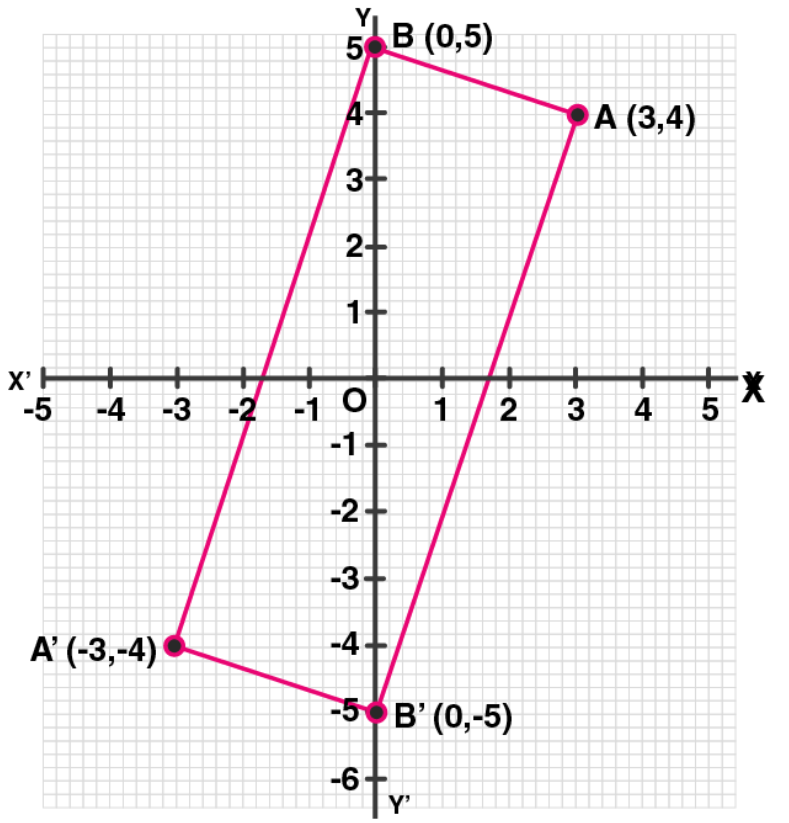# ML Aggarwal Solutions for Class 10 Maths Chapter 10 Reflection

ML Aggarwal Solutions for Class 10 Maths Chapter 10 Reflection help students understand all the crucial concepts in the chapter clearly and easily. This also develops a strong foundation in the respective subject. Referring to these solutions will improve the academic performance of students and in turn, will help them chase their desired course of study in higher classes. Here the students can download ML Aggarwal Solutions for Class 10 Maths Chapter 10 Reflection free PDF, from the link given below.

In this chapter, the students will learn about some basic concepts of coordinate geometry, understanding the concept of reflection of a point in a line and reflection of a point in a point. Further, students will be solving problems related to these concepts in the exercise. It’s suggested that when students solve problems using ML Aggarwal Solutions on a daily basis, their concept knowledge and problem-solving abilities become stronger. The solutions are provided in PDF format and are accurate and follow the latest ICSE guidelines, in order to help students score well in exams.

## ML Aggarwal Solutions for Class 10 Maths Chapter 10 :-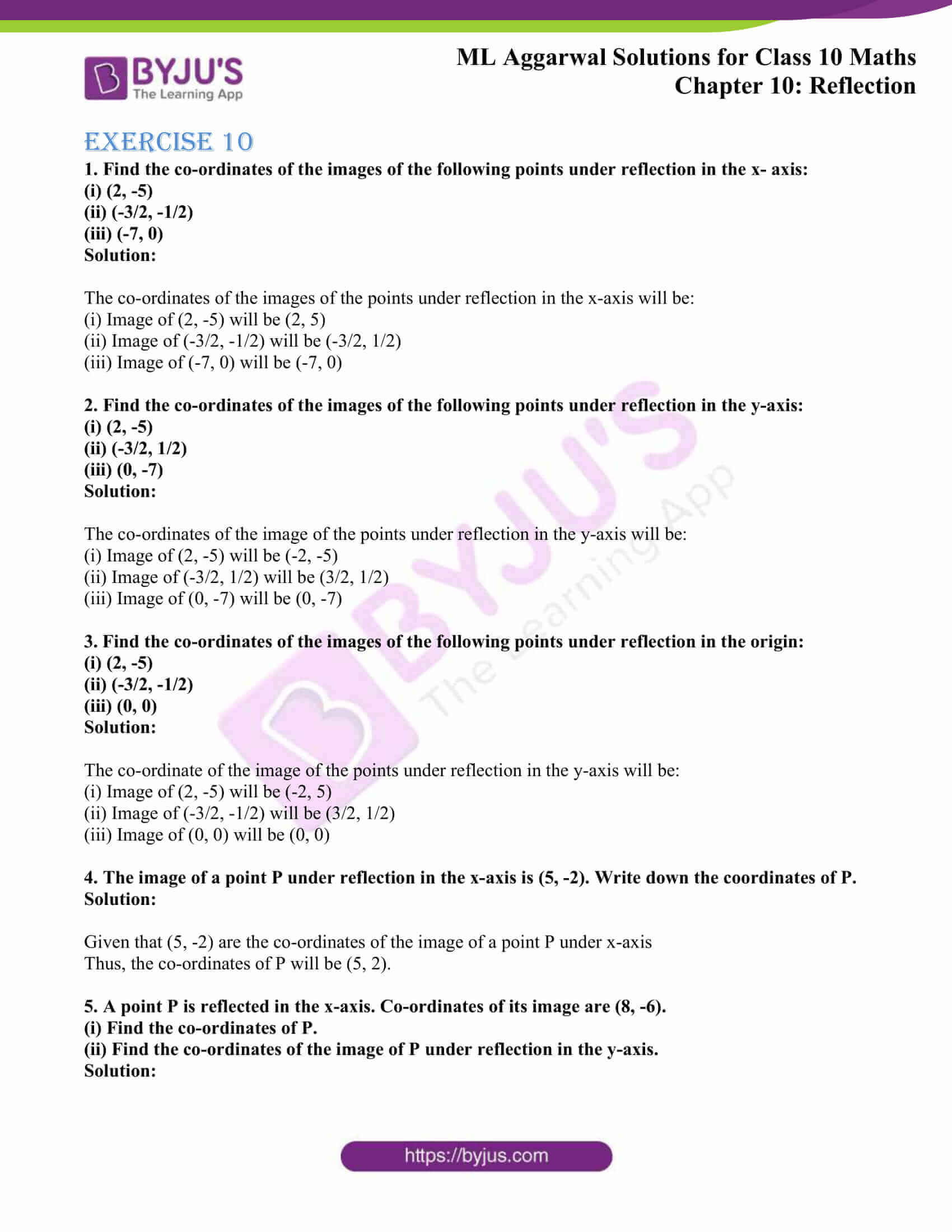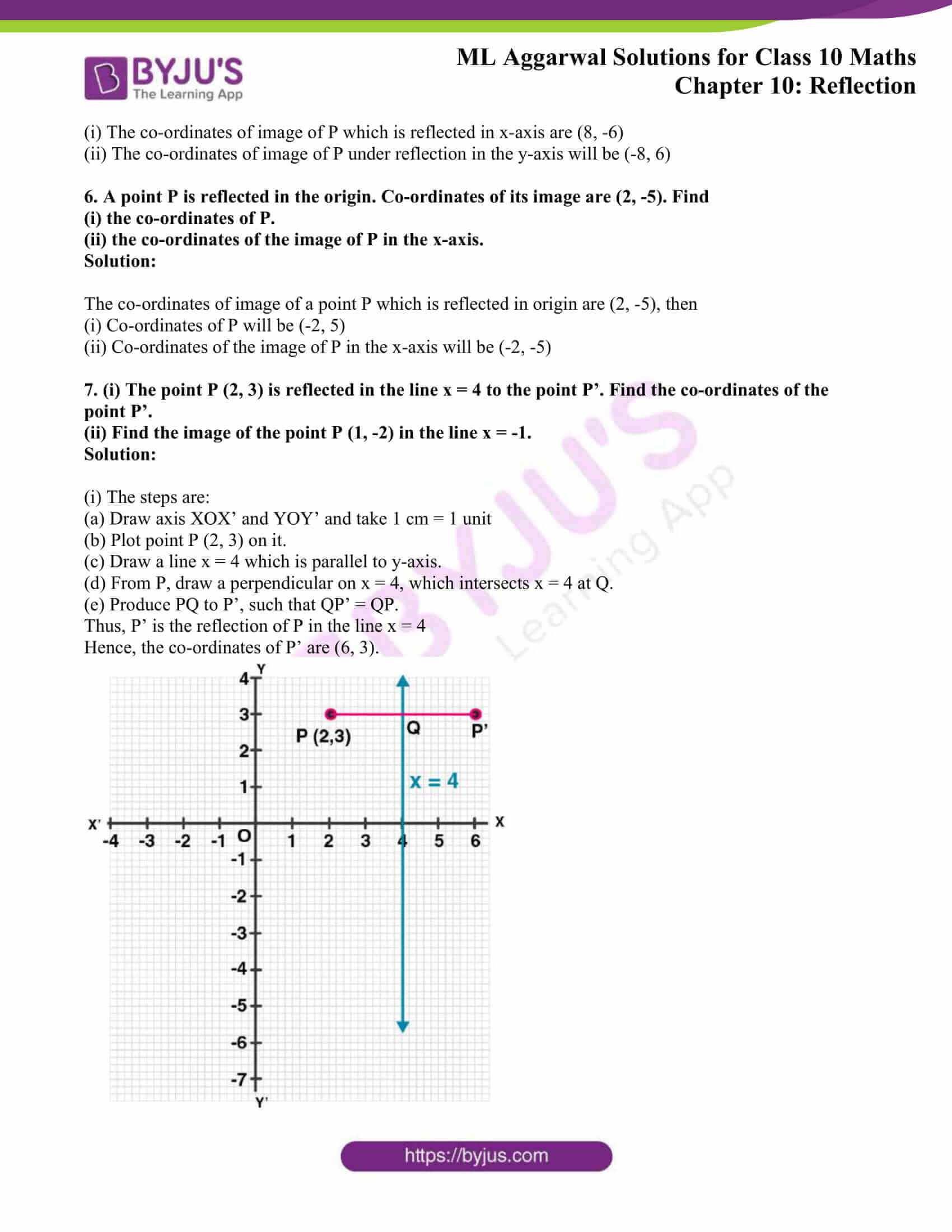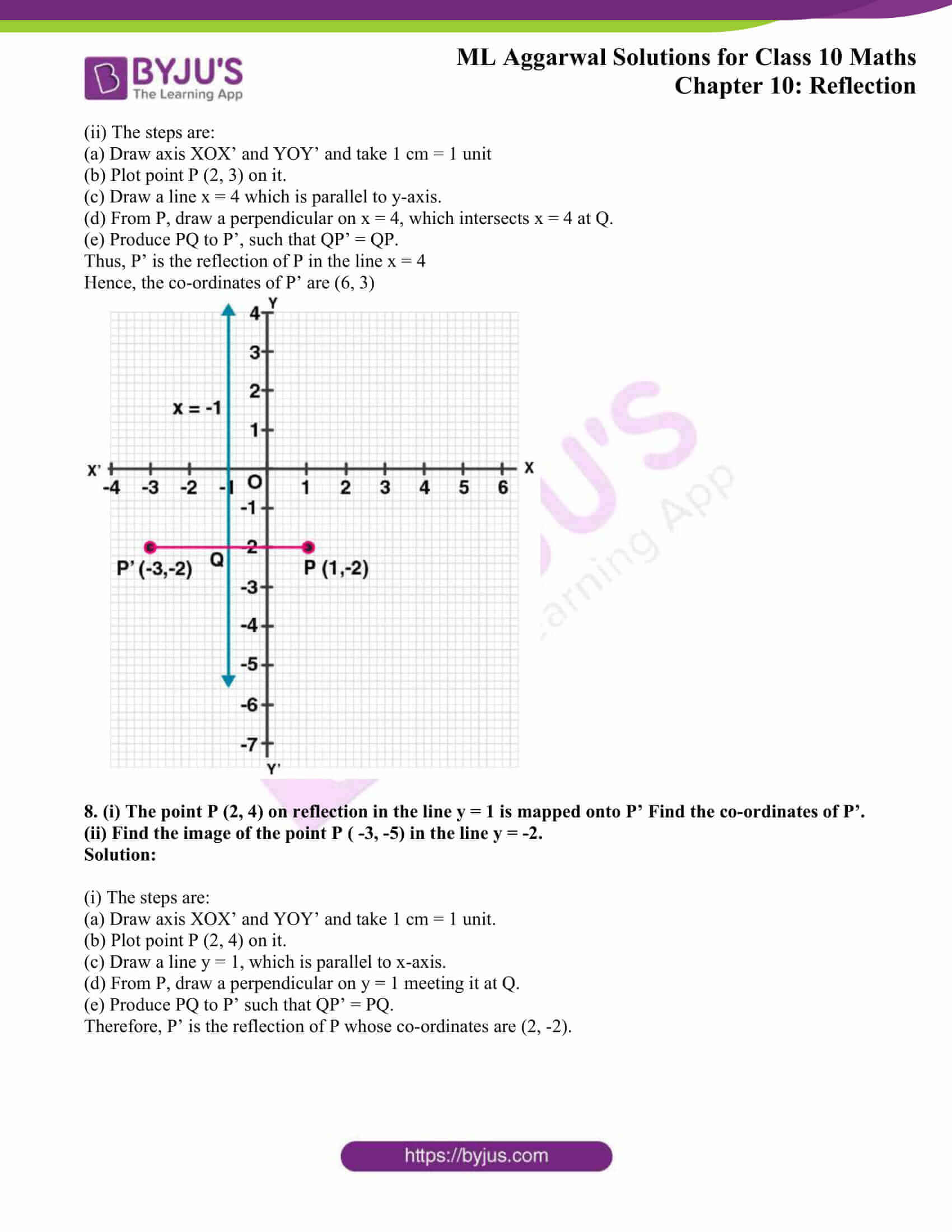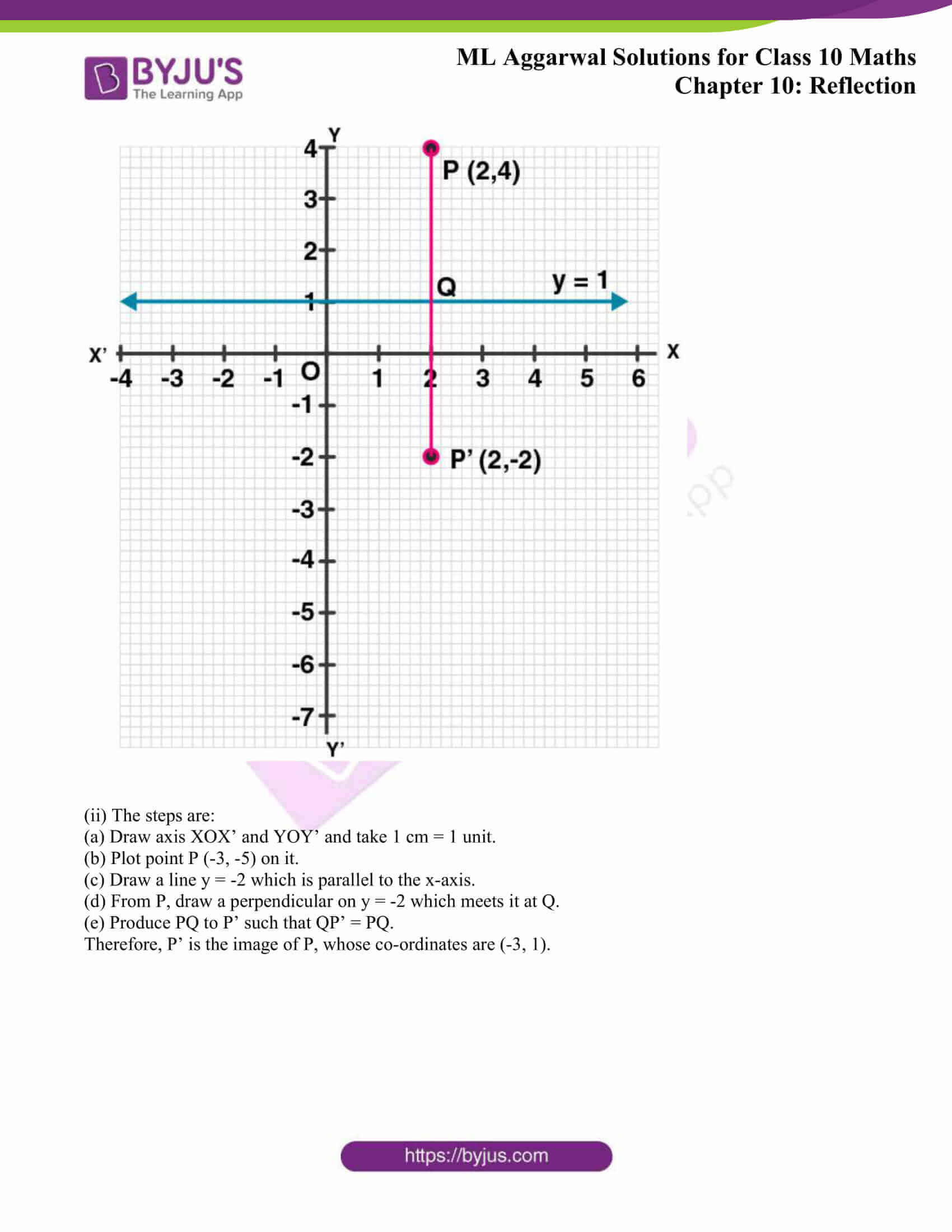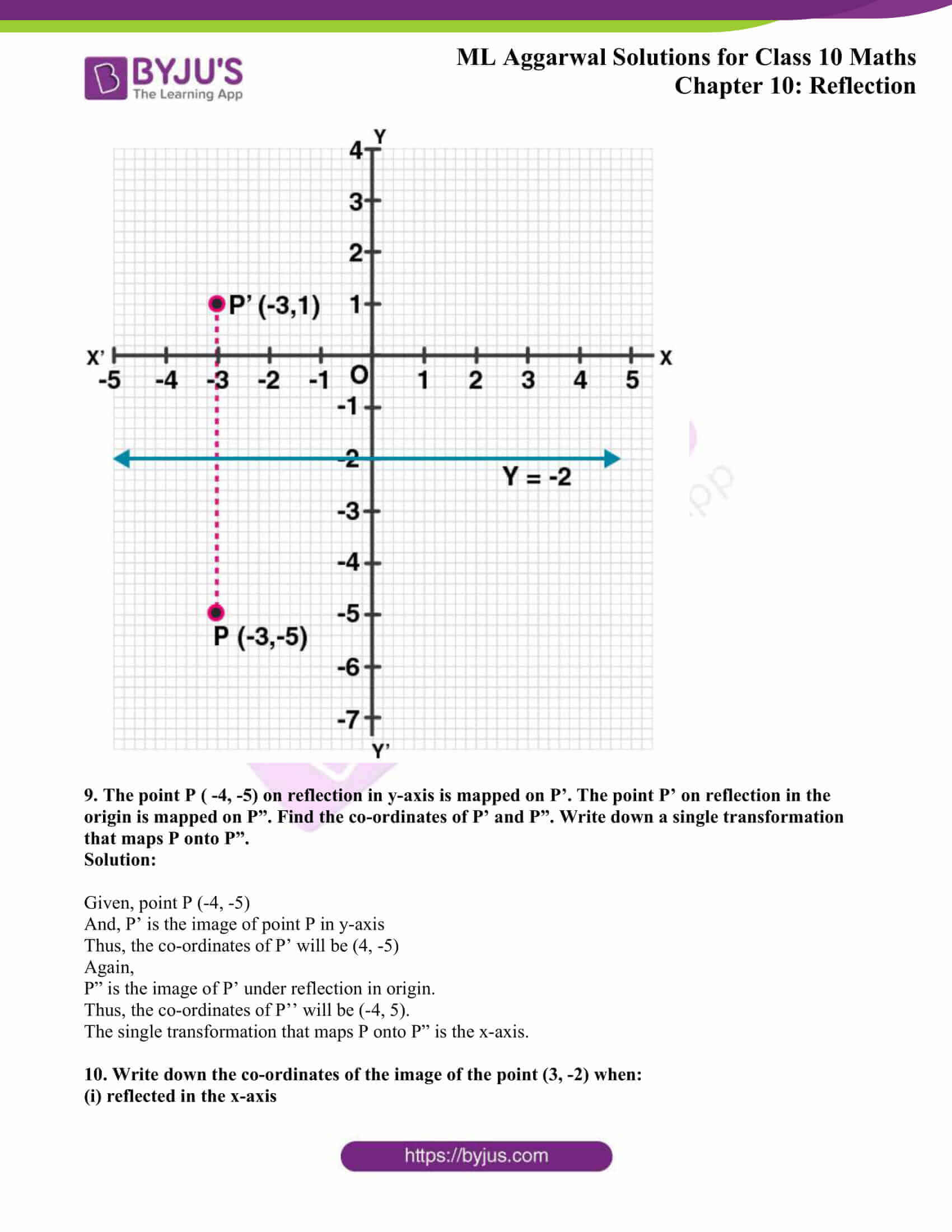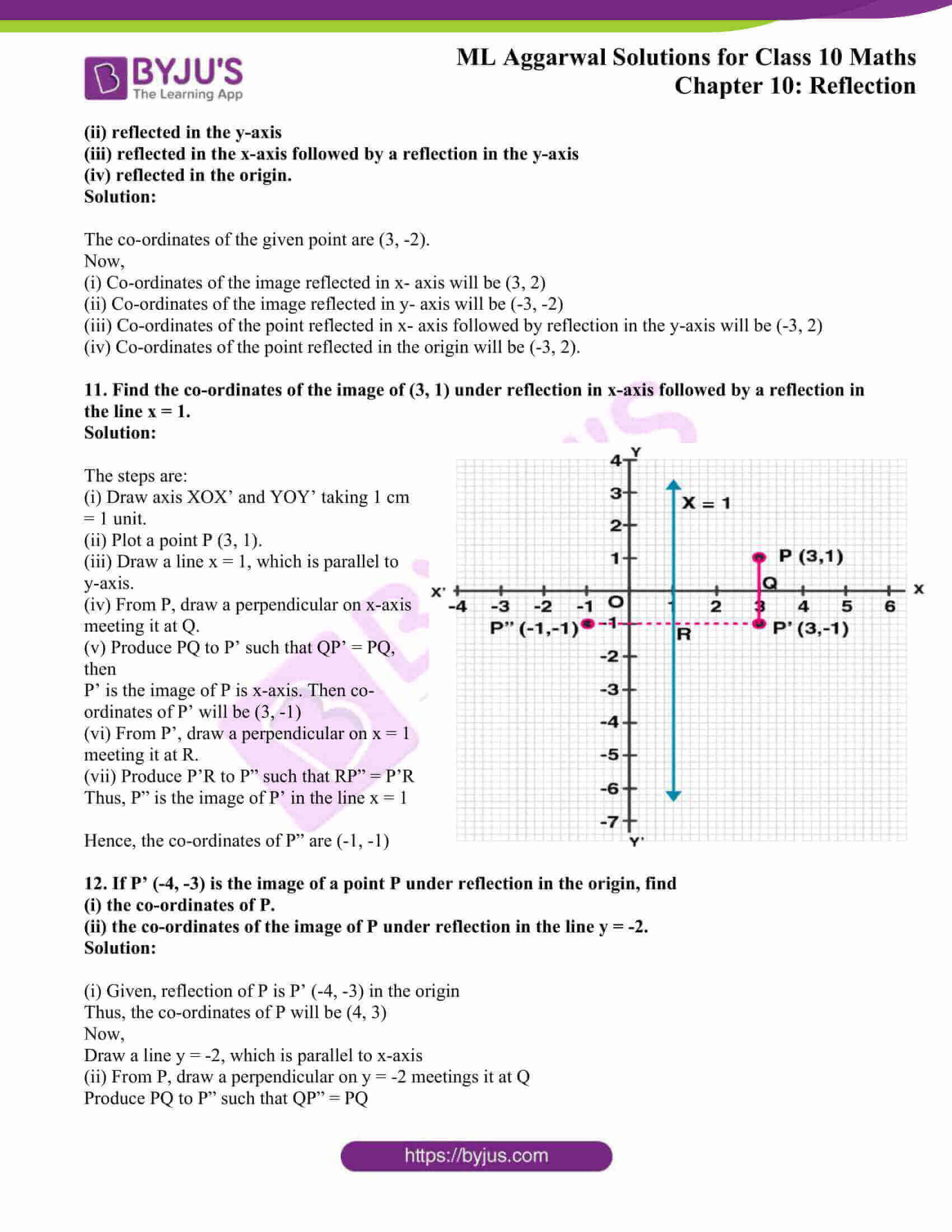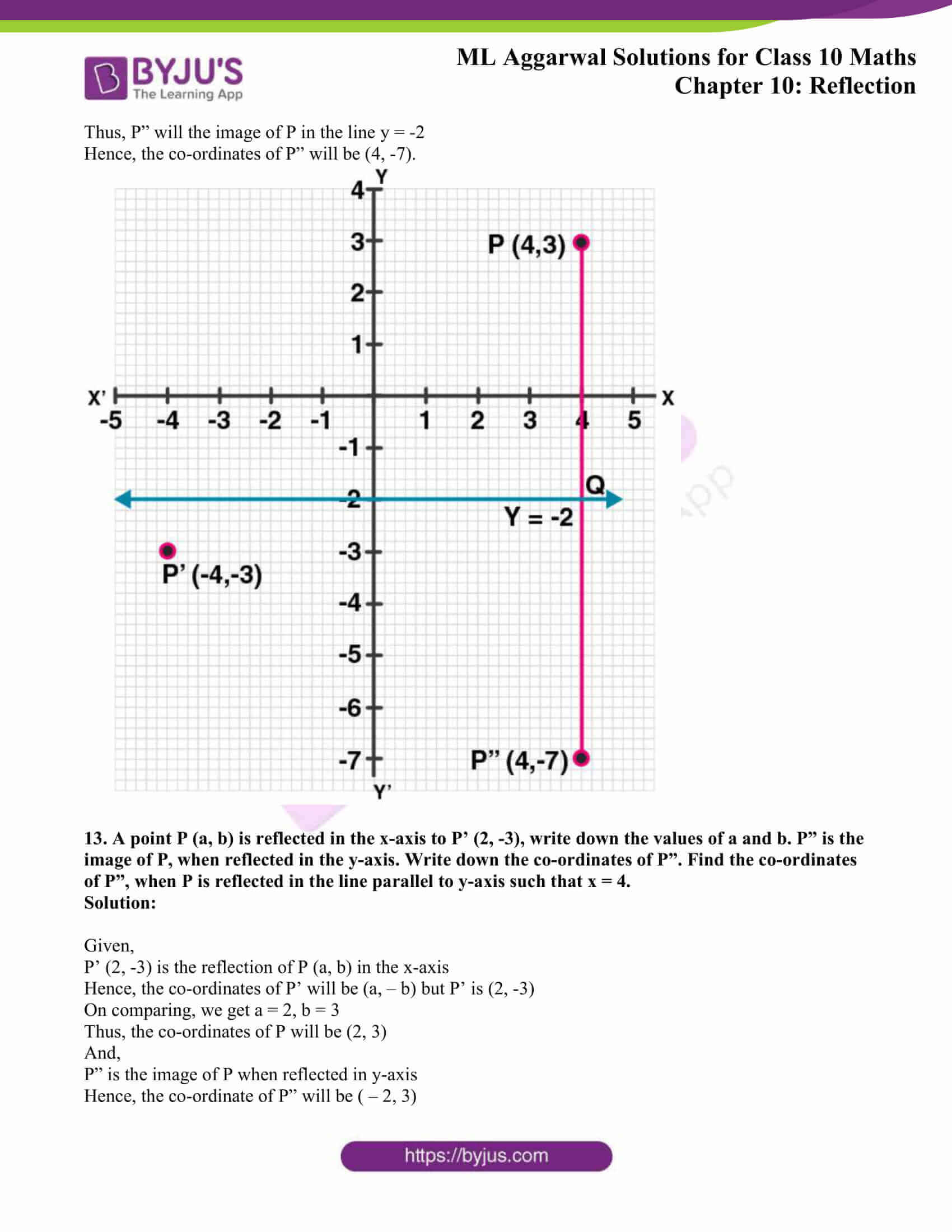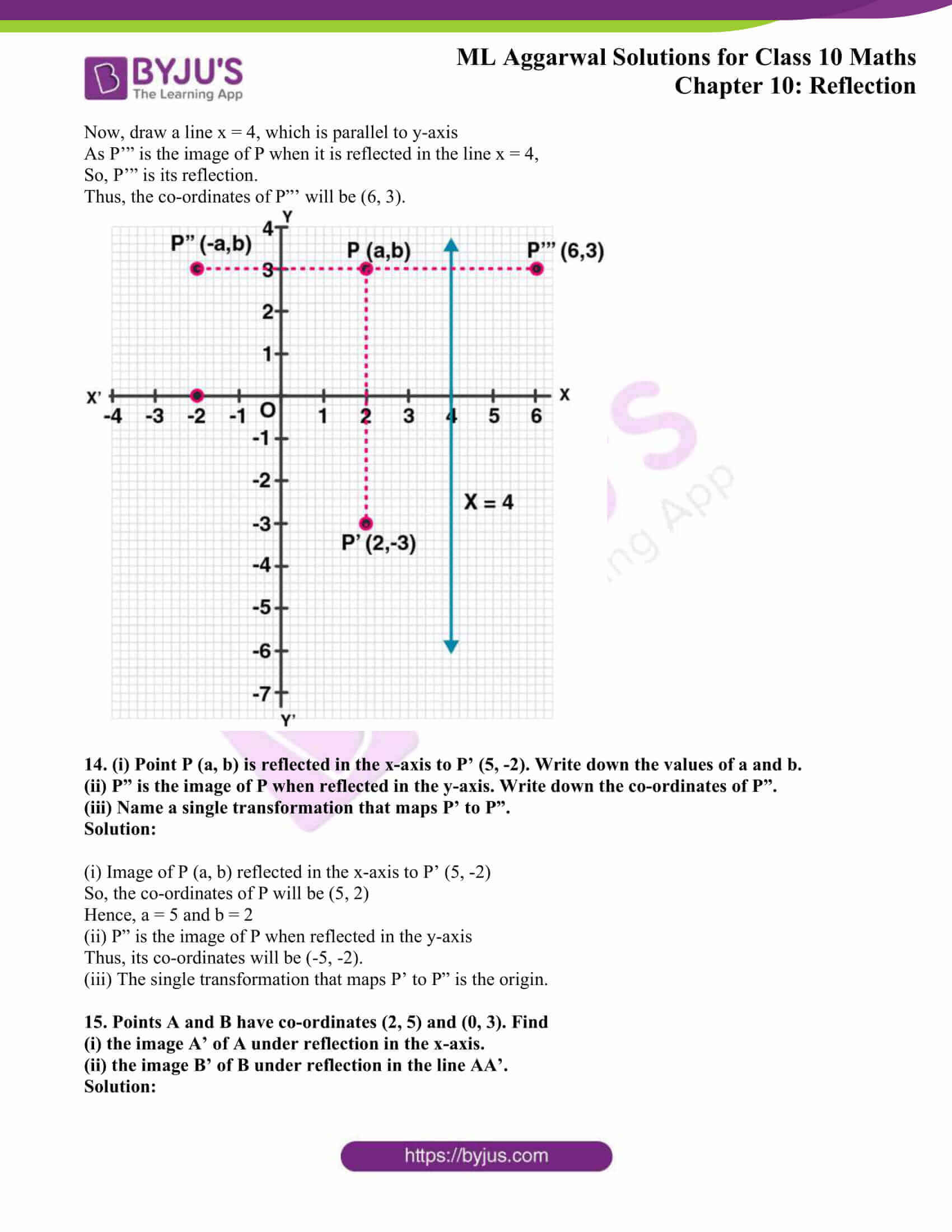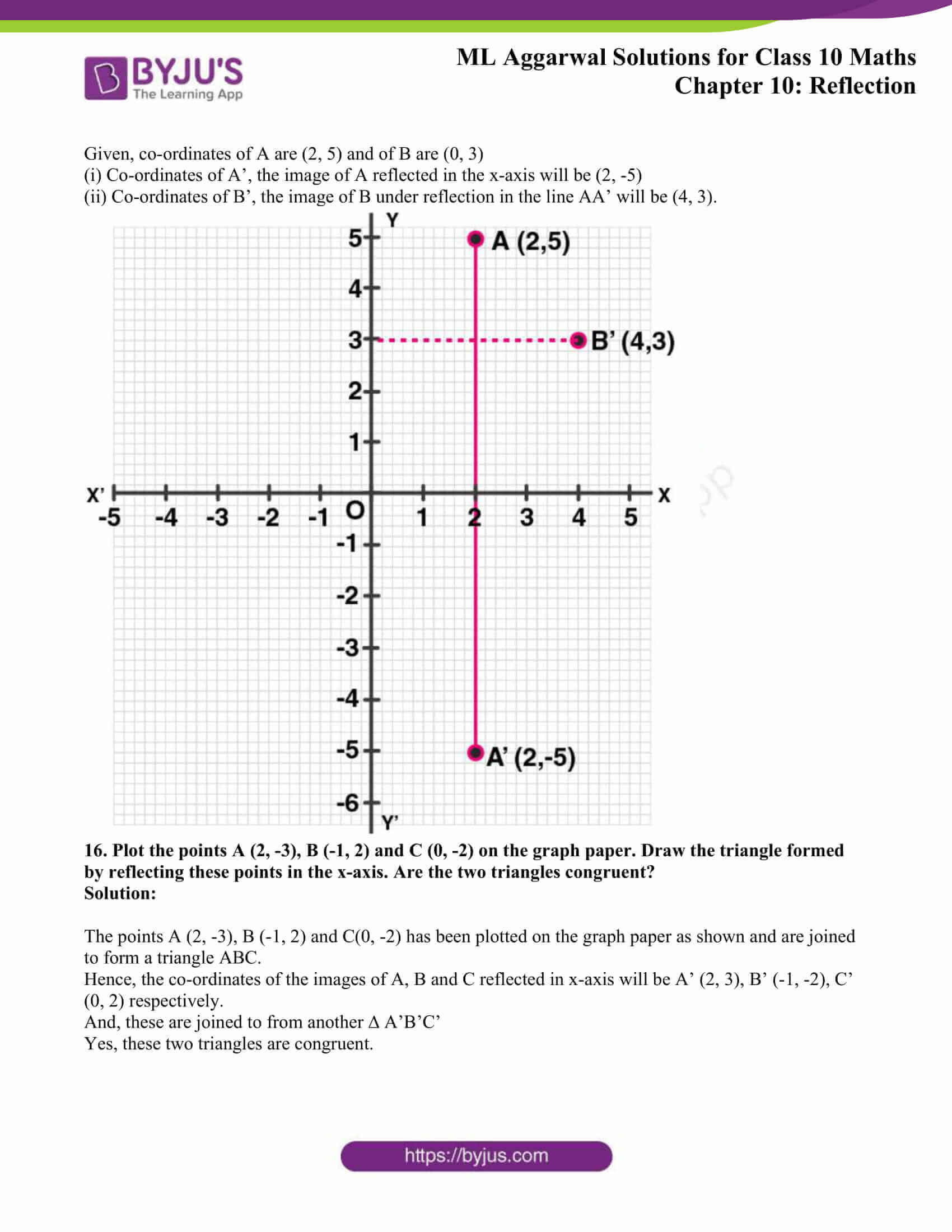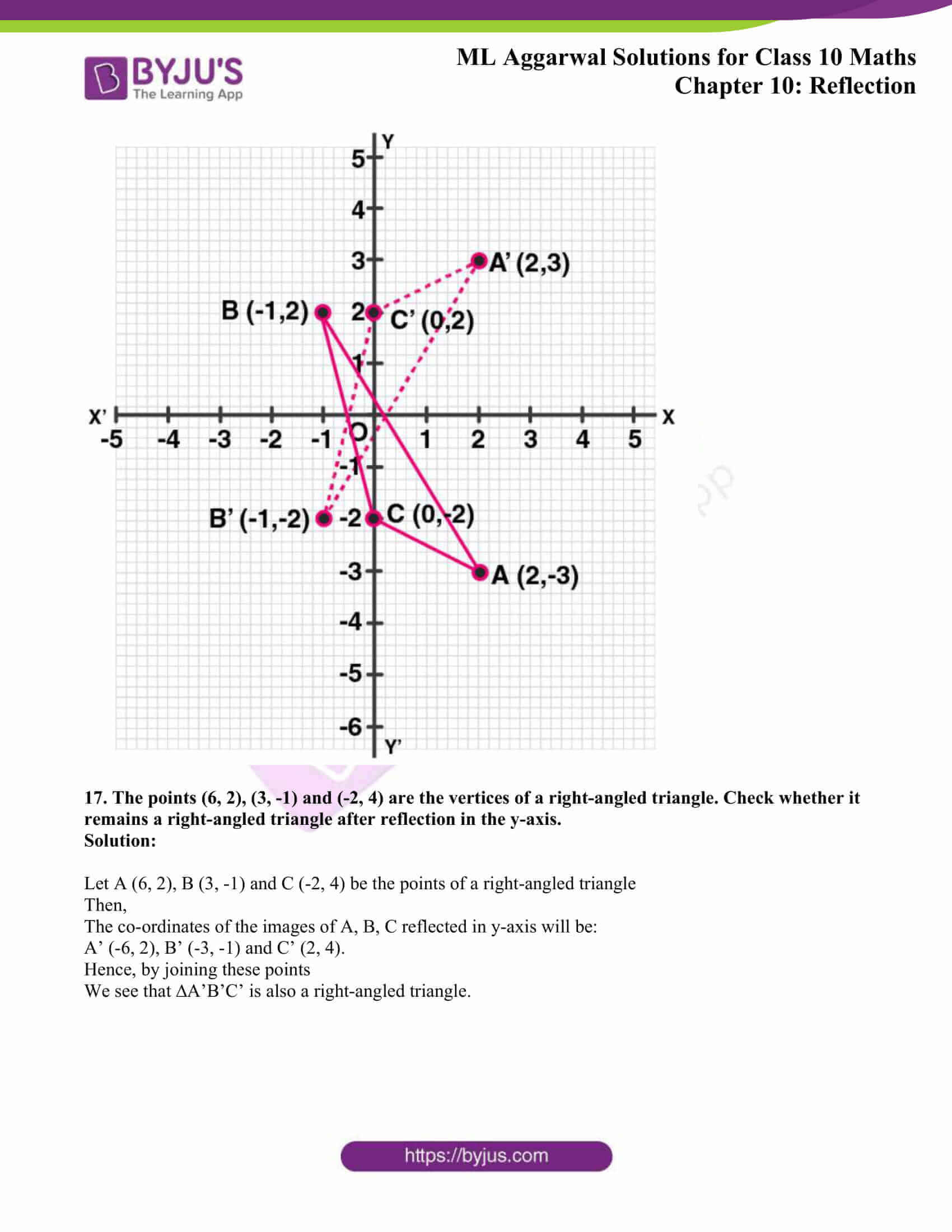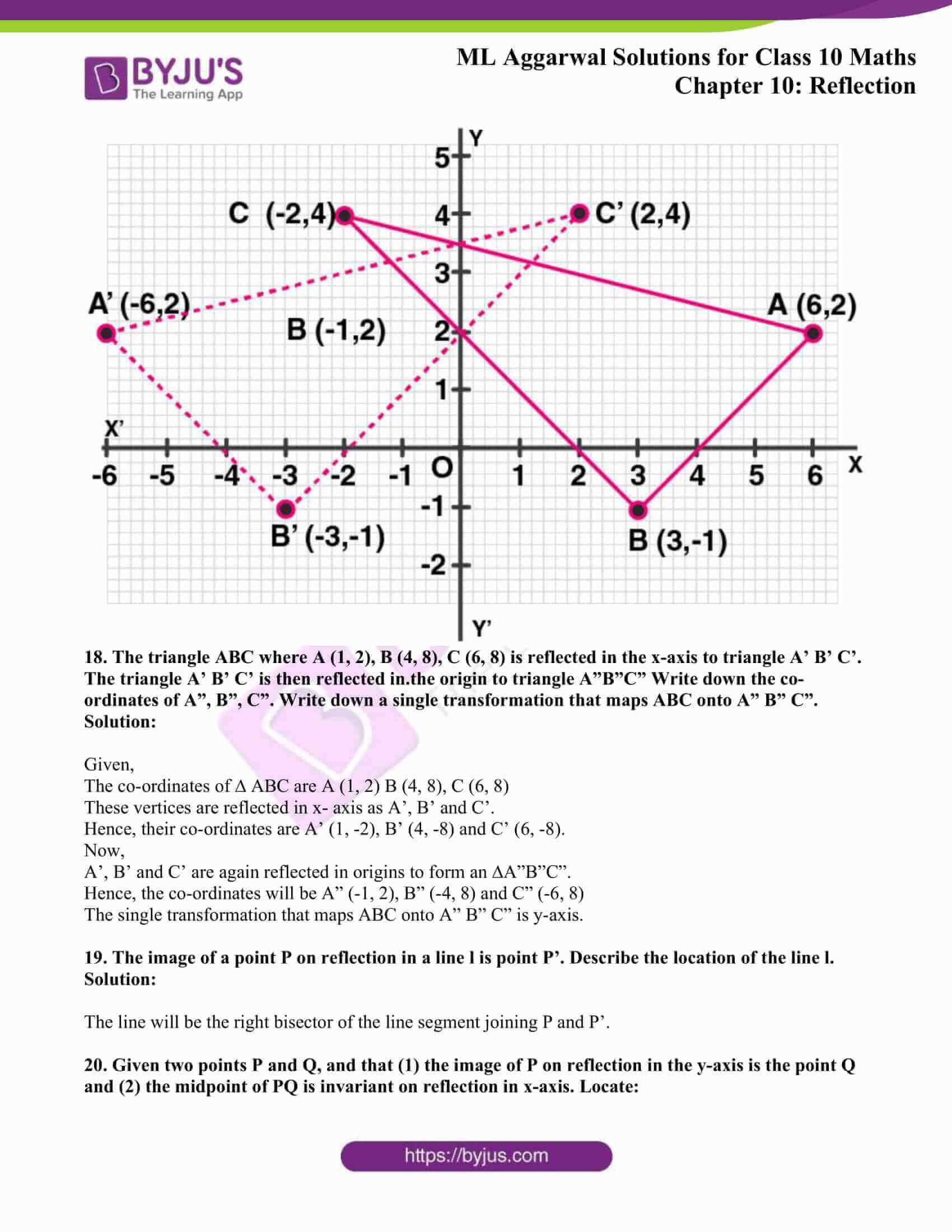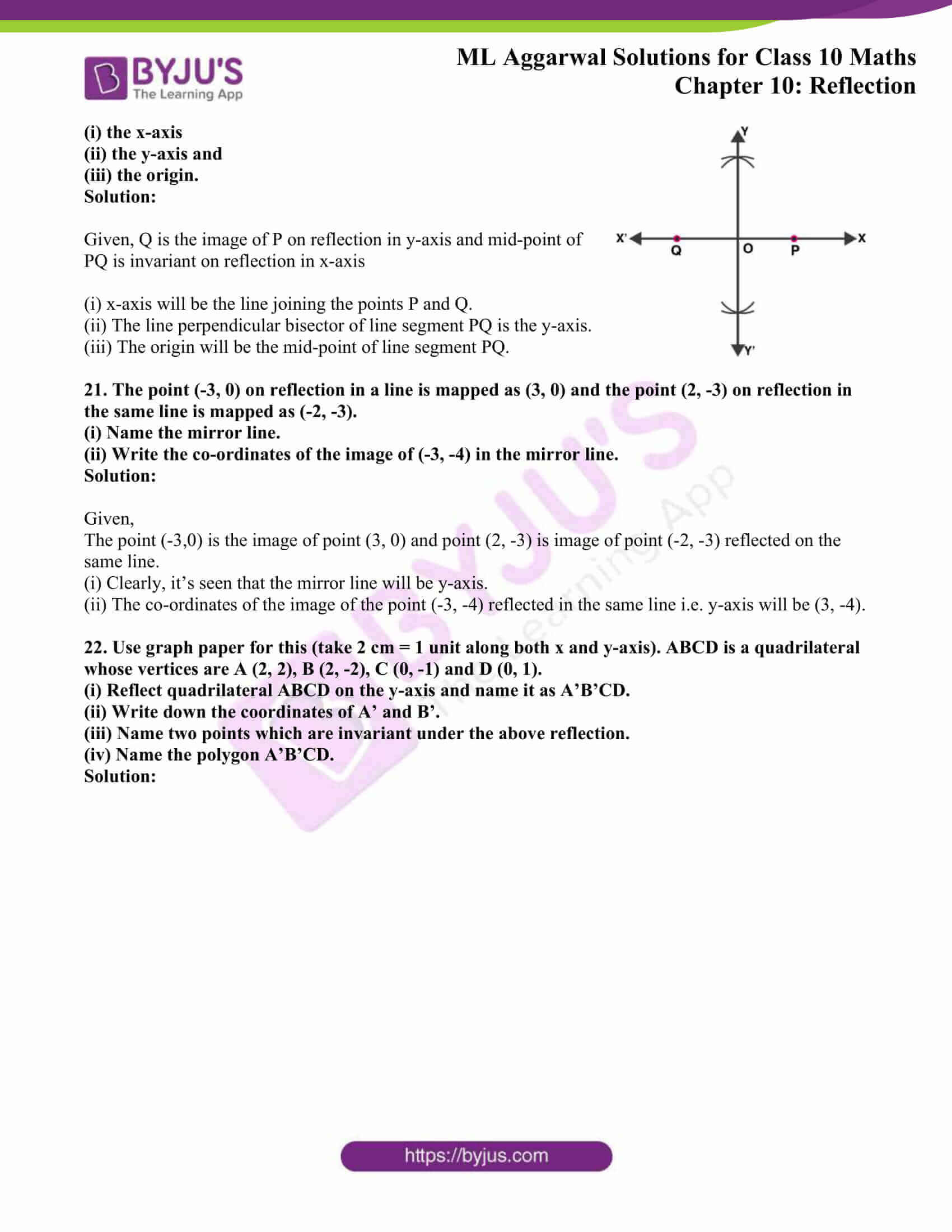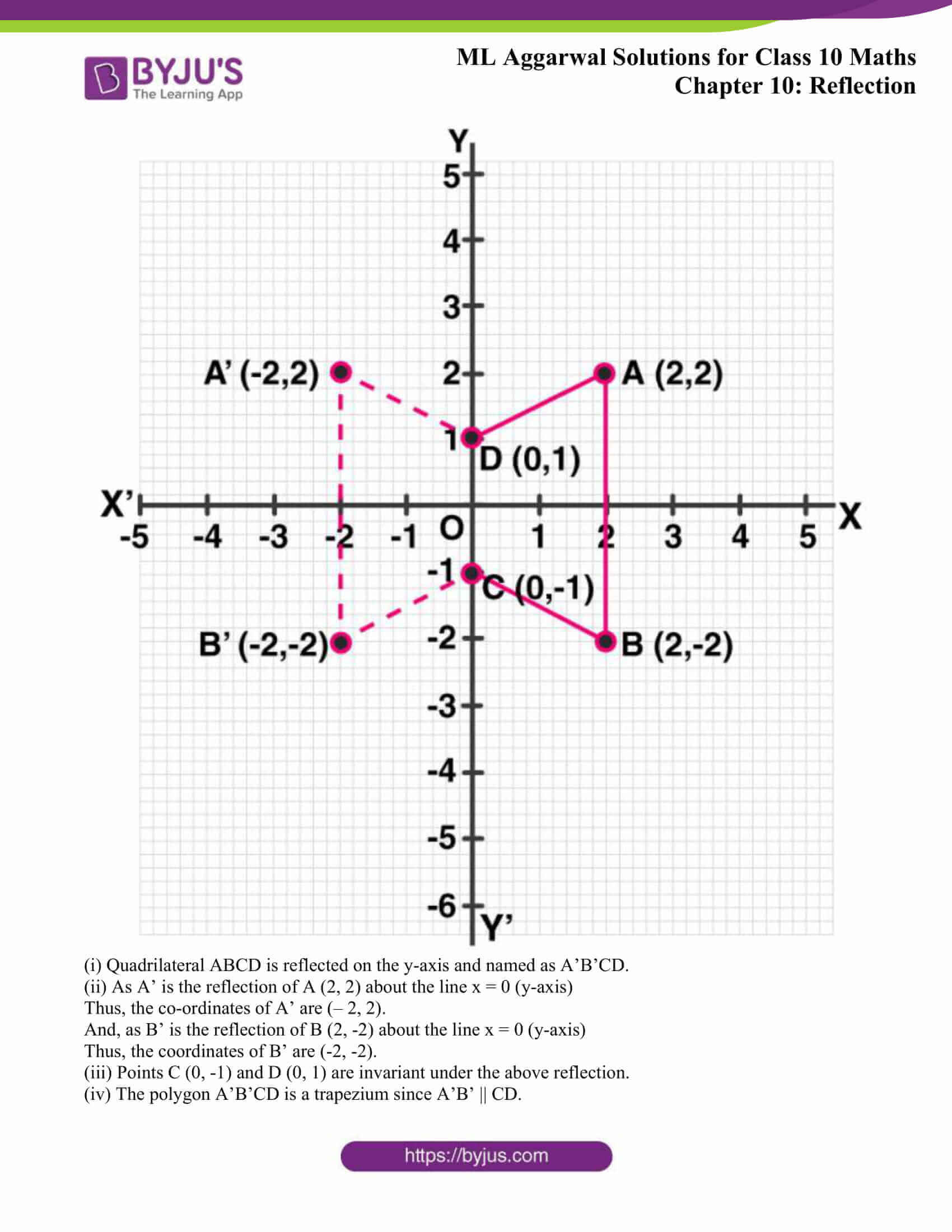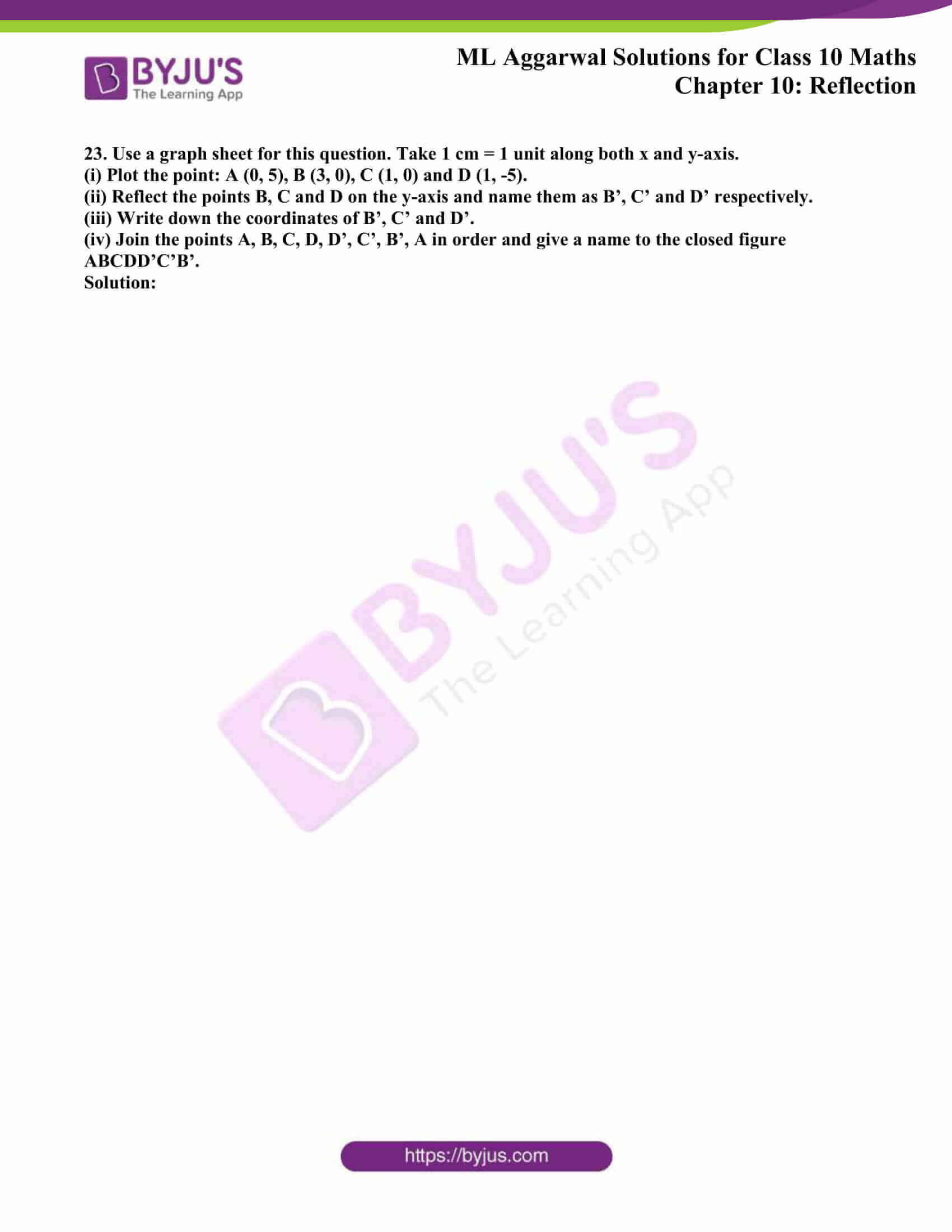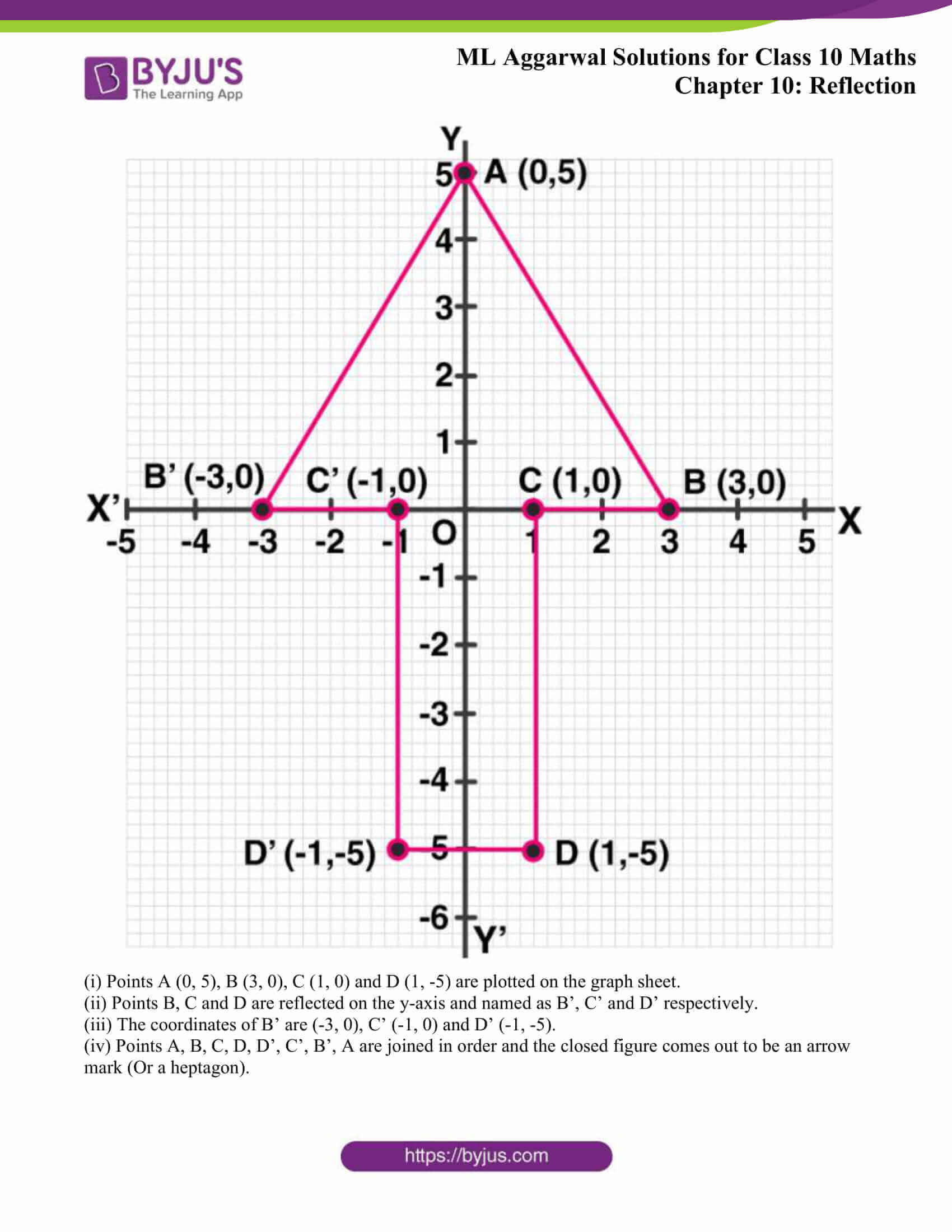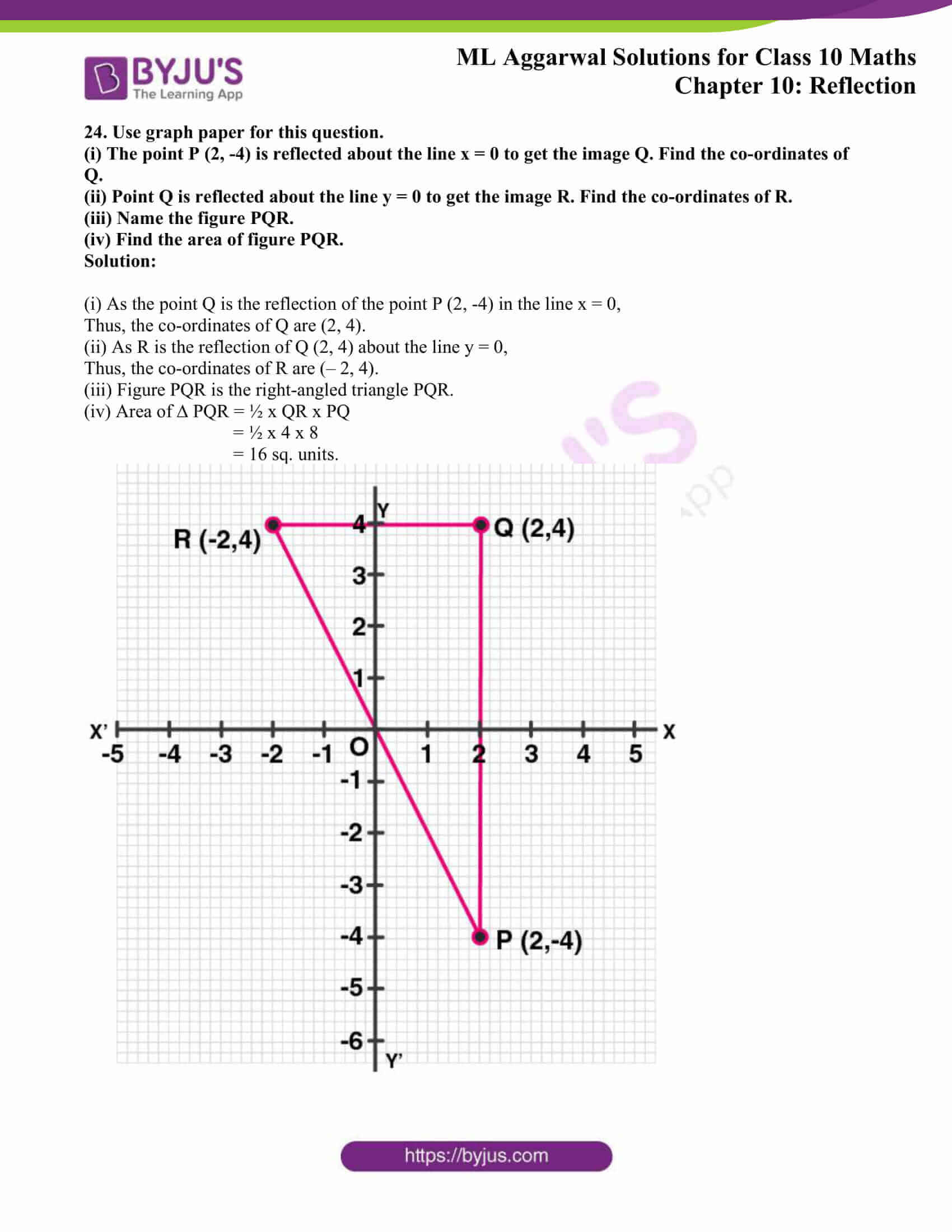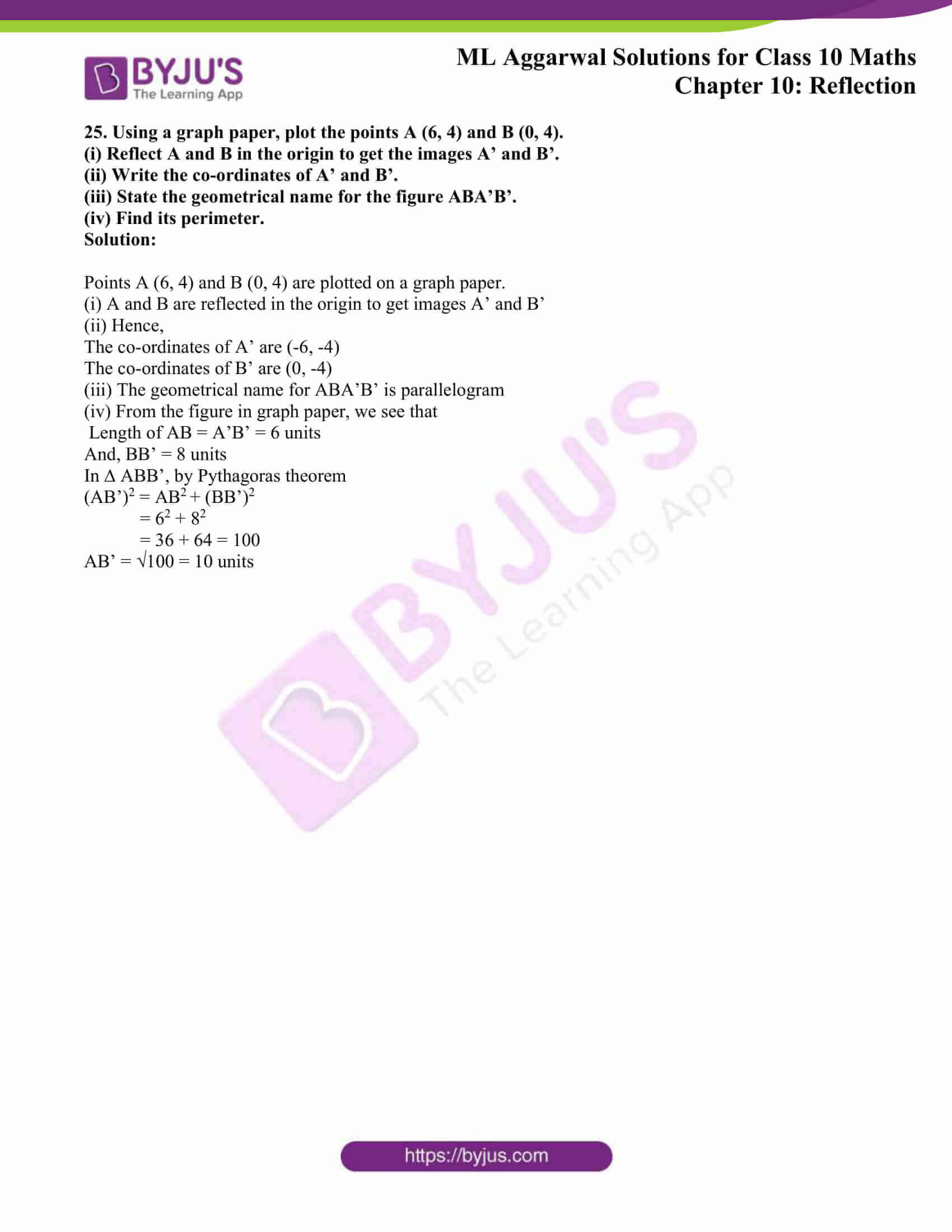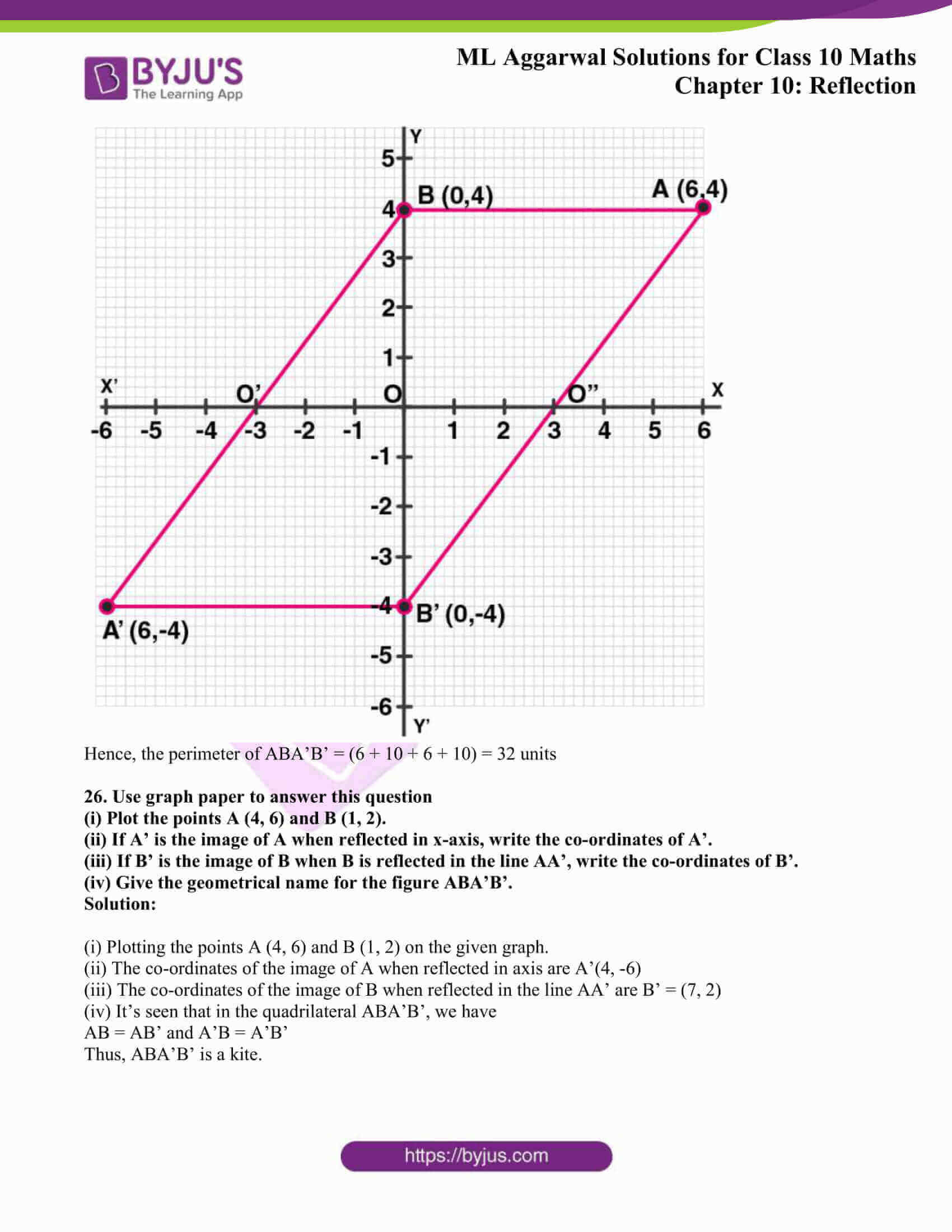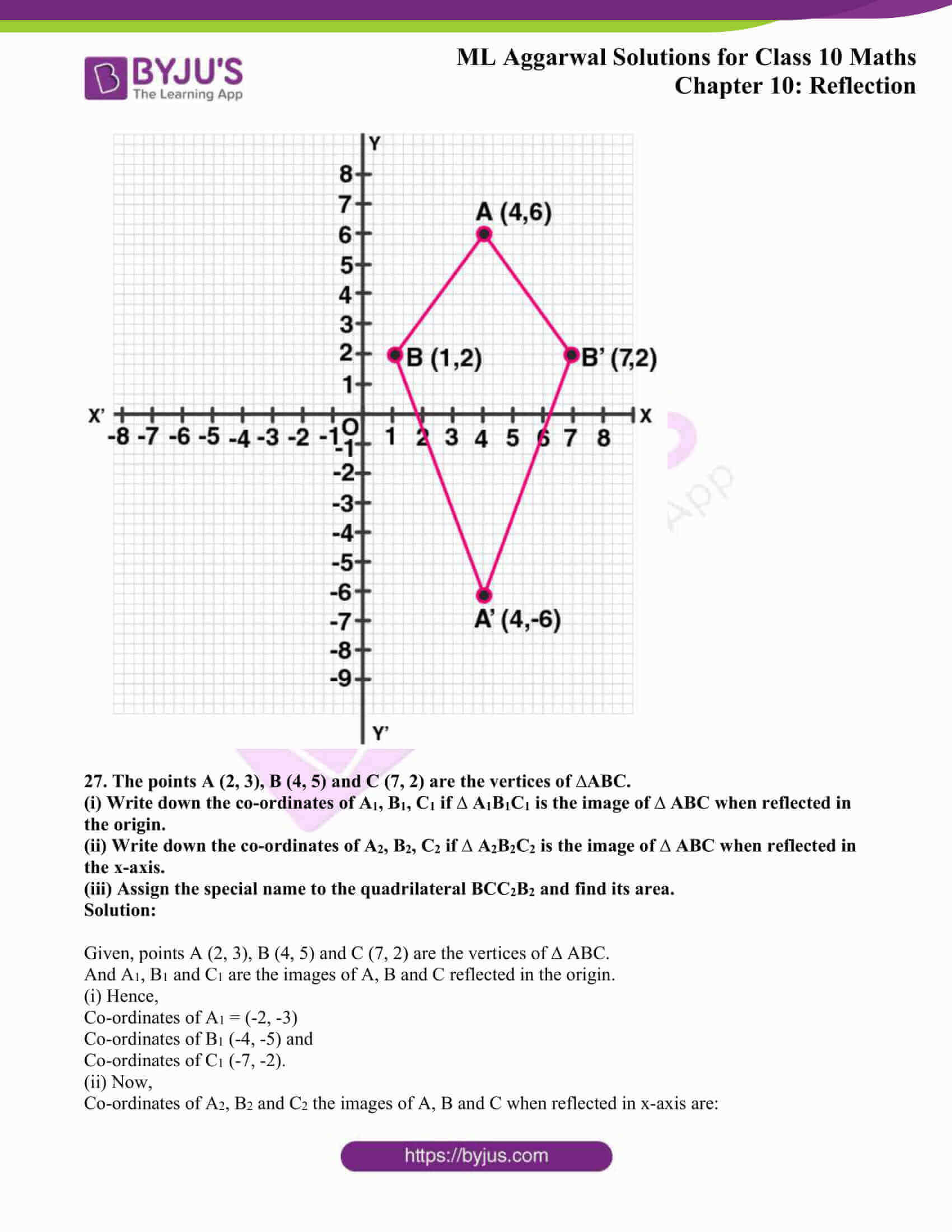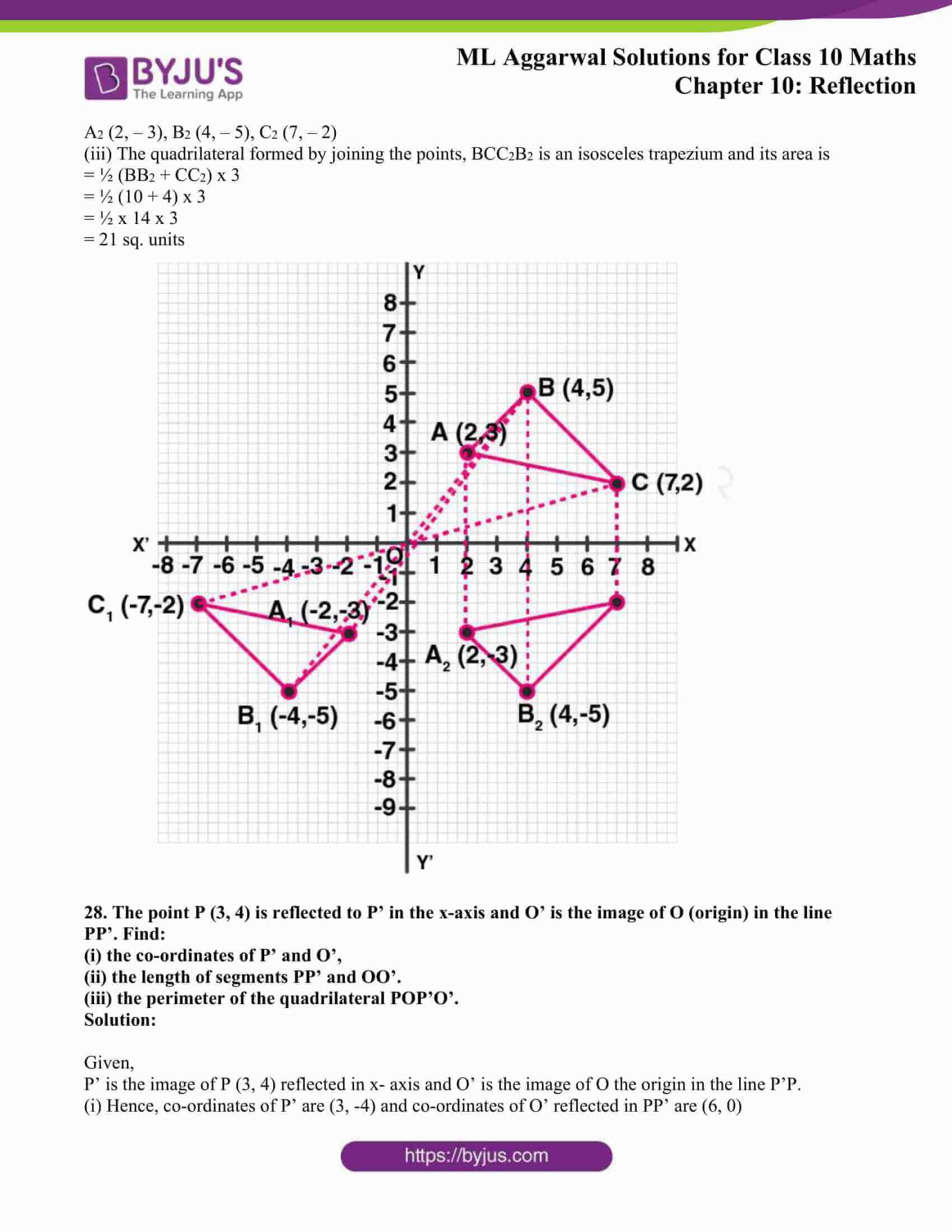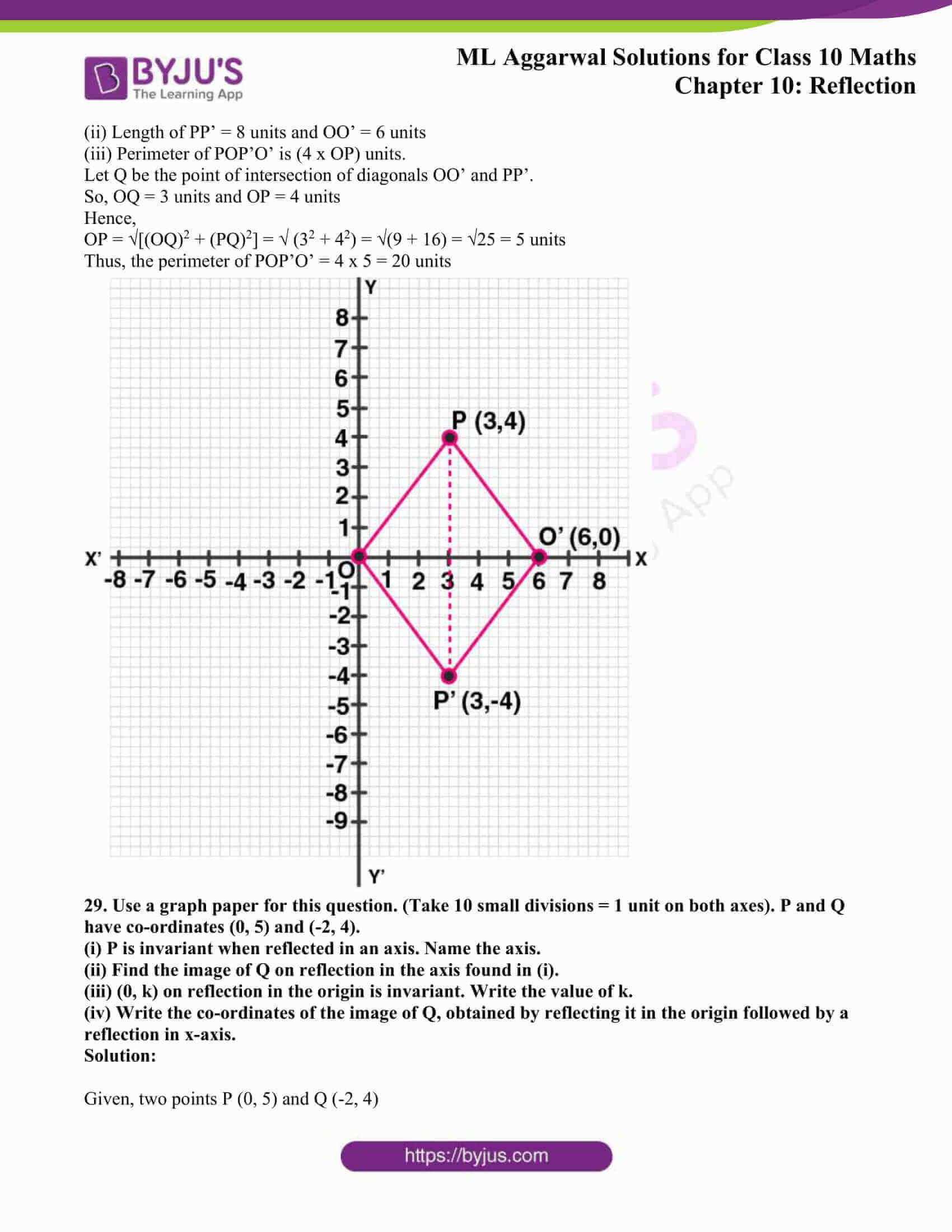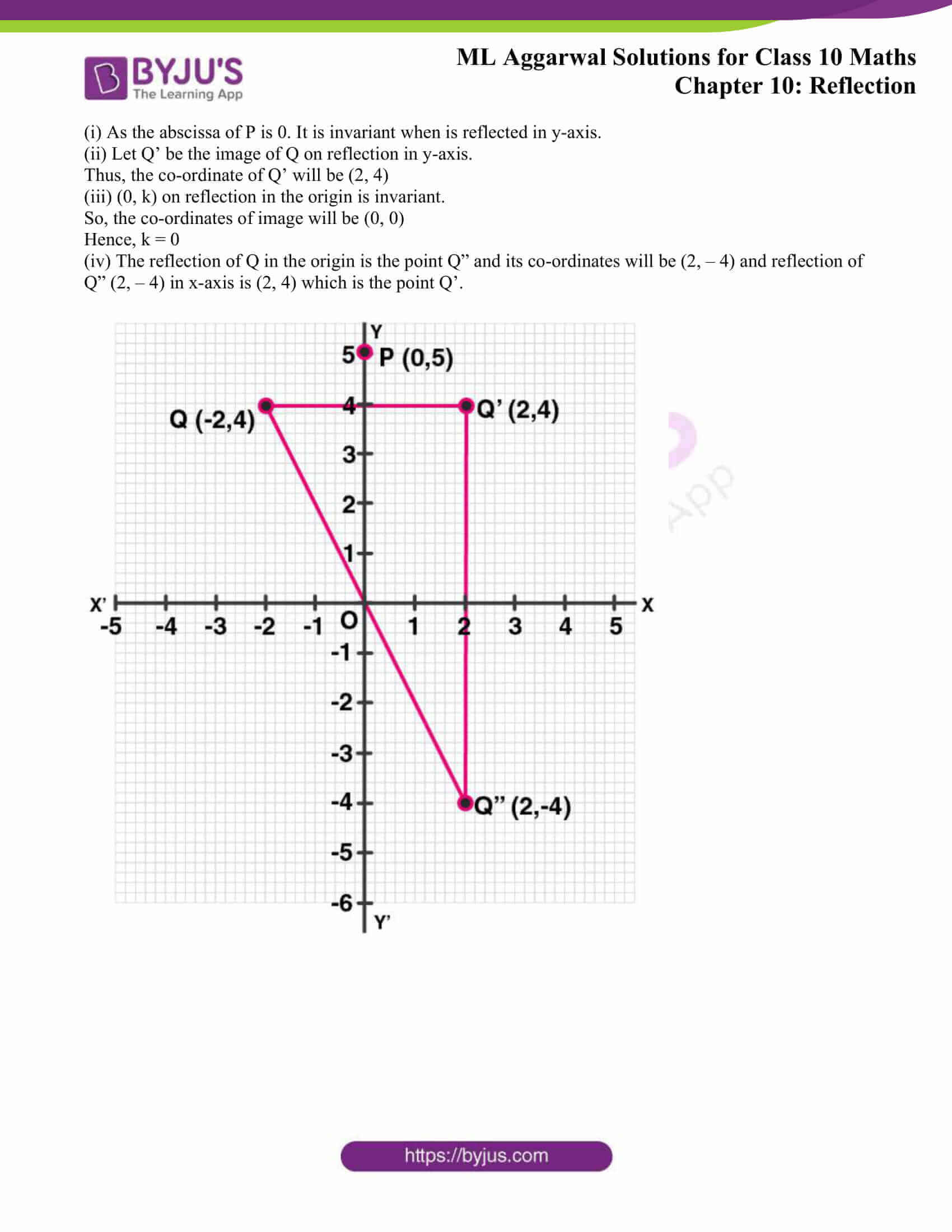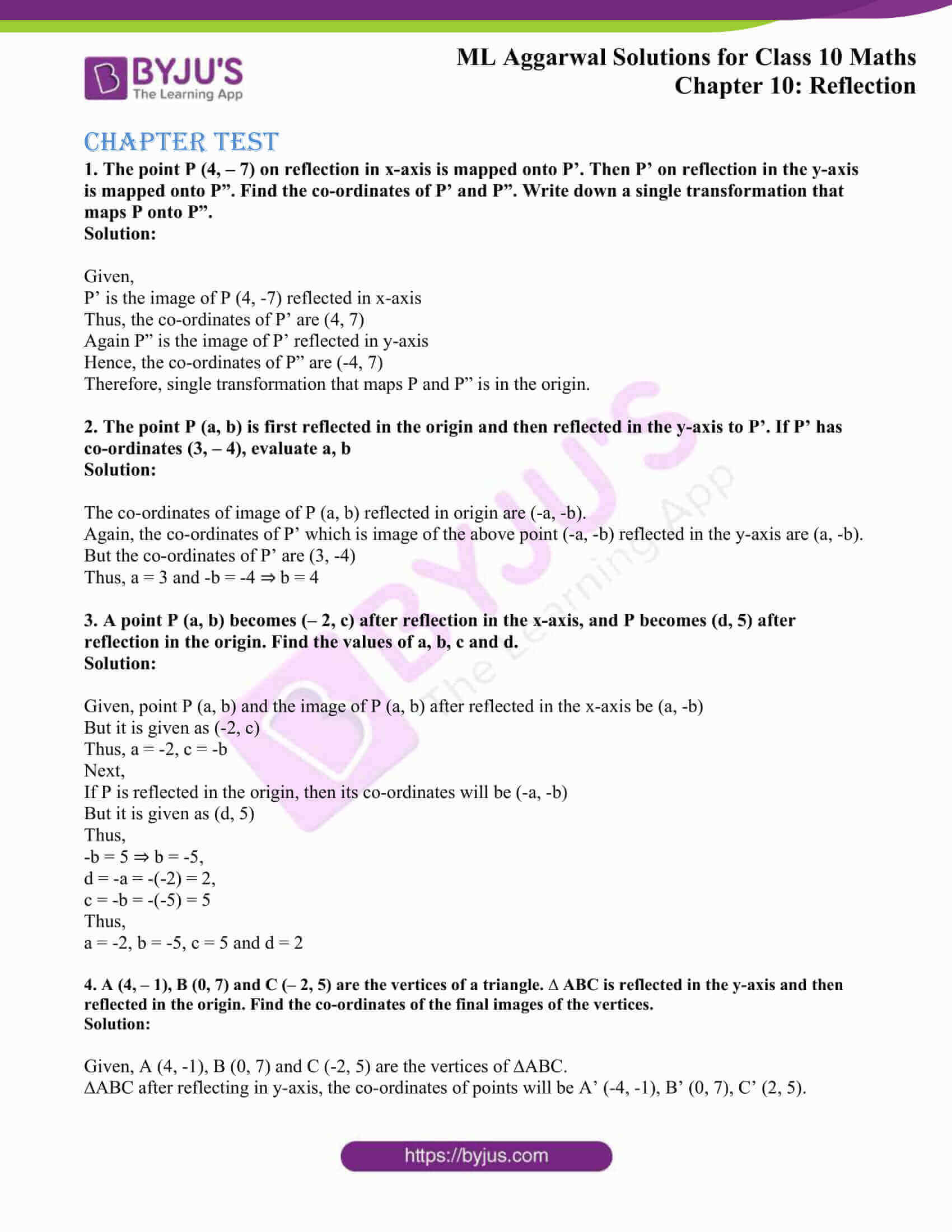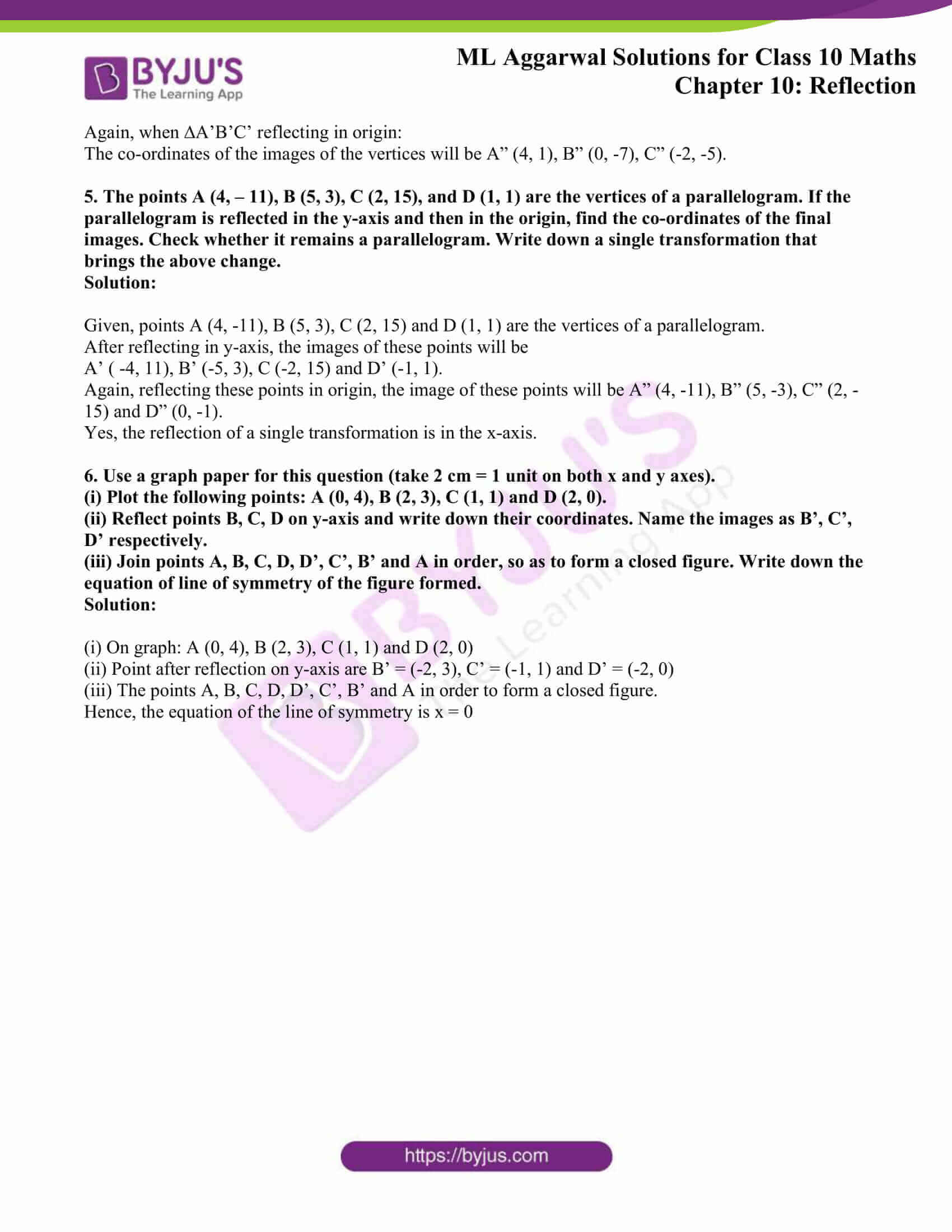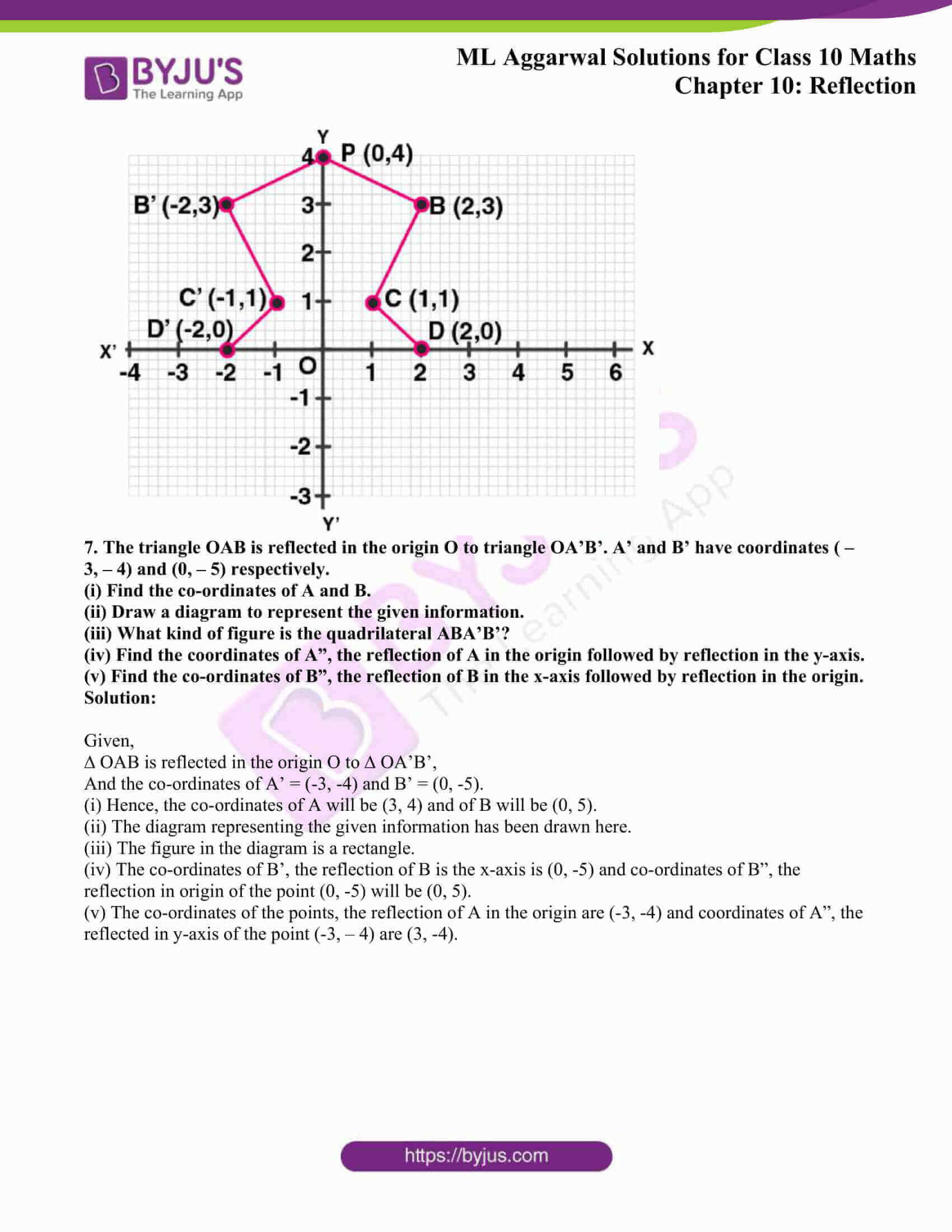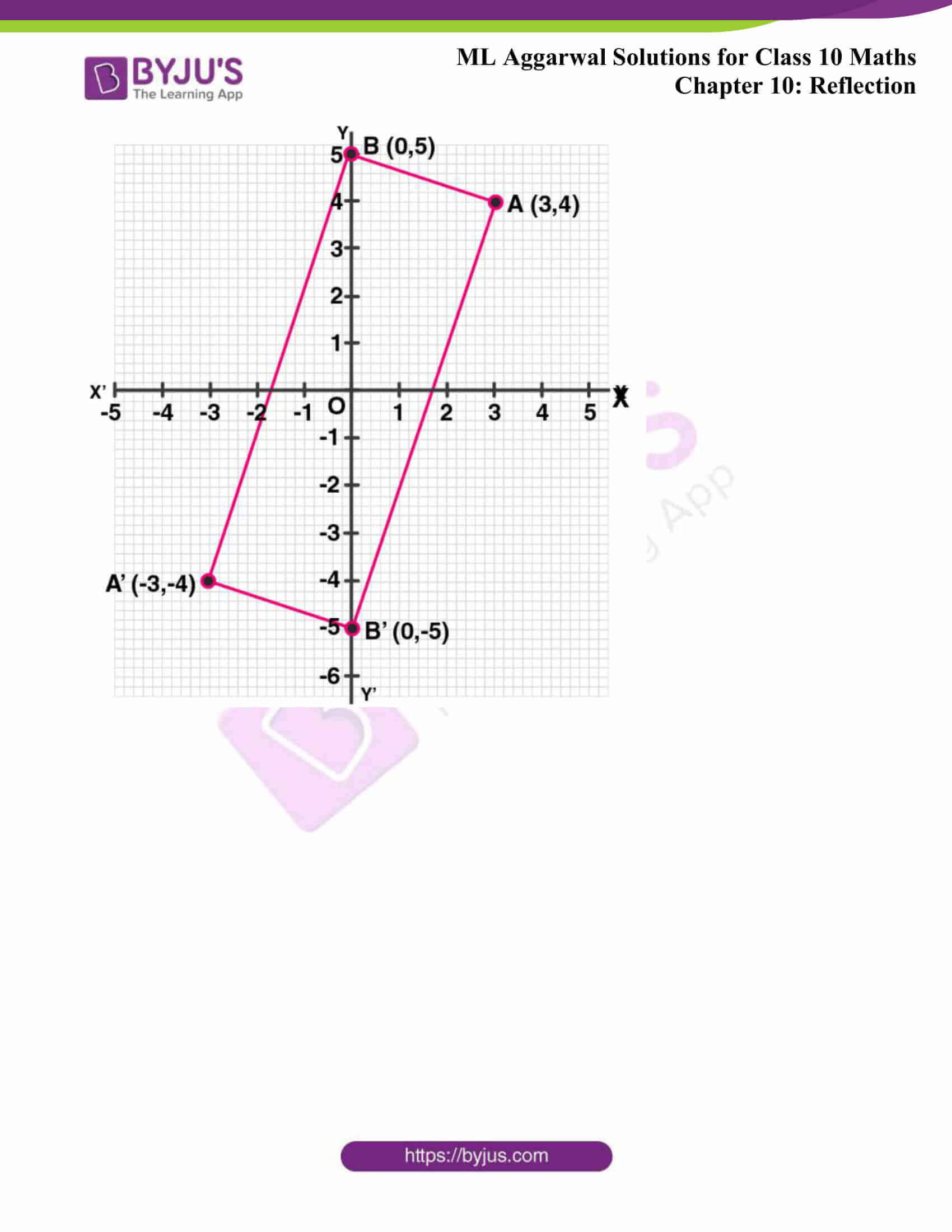## Access ML Aggarwal Solutions for Class 10 Maths Chapter 10 Reflection

Exercise 10

1. Find the co-ordinates of the images of the following points under reflection in the x- axis:
(i) (2, -5)

(ii) (-3/2, -1/2)

(iii) (-7, 0)

Solution:

The co-ordinates of the images of the points under reflection in the x-axis will be:

(i) Image of (2, -5) will be (2, 5)

(ii) Image of (-3/2, -1/2) will be (-3/2, 1/2)

(iii) Image of (-7, 0) will be (-7, 0)

2. Find the co-ordinates of the images of the following points under reflection in the y-axis:
(i) (2, -5)
(ii) (-3/2, 1/2)
(iii) (0, -7)
Solution:

The co-ordinates of the image of the points under reflection in the y-axis will be:

(i) Image of (2, -5) will be (-2, -5)

(ii) Image of (-3/2, 1/2) will be (3/2, 1/2)

(iii) Image of (0, -7) will be (0, -7)

3. Find the co-ordinates of the images of the following points under reflection in the origin:
(i) (2, -5)
(ii) (-3/2, -1/2)
(iii) (0, 0)
Solution:

The co-ordinate of the image of the points under reflection in the y-axis will be:

(i) Image of (2, -5) will be (-2, 5)

(ii) Image of (-3/2, -1/2) will be (3/2, 1/2)

(iii) Image of (0, 0) will be (0, 0)

4. The image of a point P under reflection in the x-axis is (5, -2). Write down the coordinates of P.
Solution:

Given that (5, -2) are the co-ordinates of the image of a point P under x-axis

Thus, the co-ordinates of P will be (5, 2).

5. A point P is reflected in the x-axis. Co-ordinates of its image are (8, -6).
(i) Find the co-ordinates of P.
(ii) Find the co-ordinates of the image of P under reflection in the y-axis.
Solution:

(i) The co-ordinates of image of P which is reflected in x-axis are (8, -6)

(ii) The co-ordinates of image of P under reflection in the y-axis will be (-8, 6)

6. A point P is reflected in the origin. Co-ordinates of its image are (2, -5). Find
(i) the co-ordinates of P.
(ii) the co-ordinates of the image of P in the x-axis.
Solution:

The co-ordinates of image of a point P which is reflected in origin are (2, -5), then

(i) Co-ordinates of P will be (-2, 5)

(ii) Co-ordinates of the image of P in the x-axis will be (-2, -5)

7. (i) The point P (2, 3) is reflected in the line x = 4 to the point P’. Find the co-ordinates of the point P’.
(ii) Find the image of the point P (1, -2) in the line x = -1.
Solution:

(i) The steps are:

(a) Draw axis XOX’ and YOY’ and take 1 cm = 1 unit

(b) Plot point P (2, 3) on it.

(c) Draw a line x = 4 which is parallel to y-axis.

(d) From P, draw a perpendicular on x = 4, which intersects x = 4 at Q.

(e) Produce PQ to P’, such that QP’ = QP.

Thus, P’ is the reflection of P in the line x = 4

Hence, the co-ordinates of P’ are (6, 3).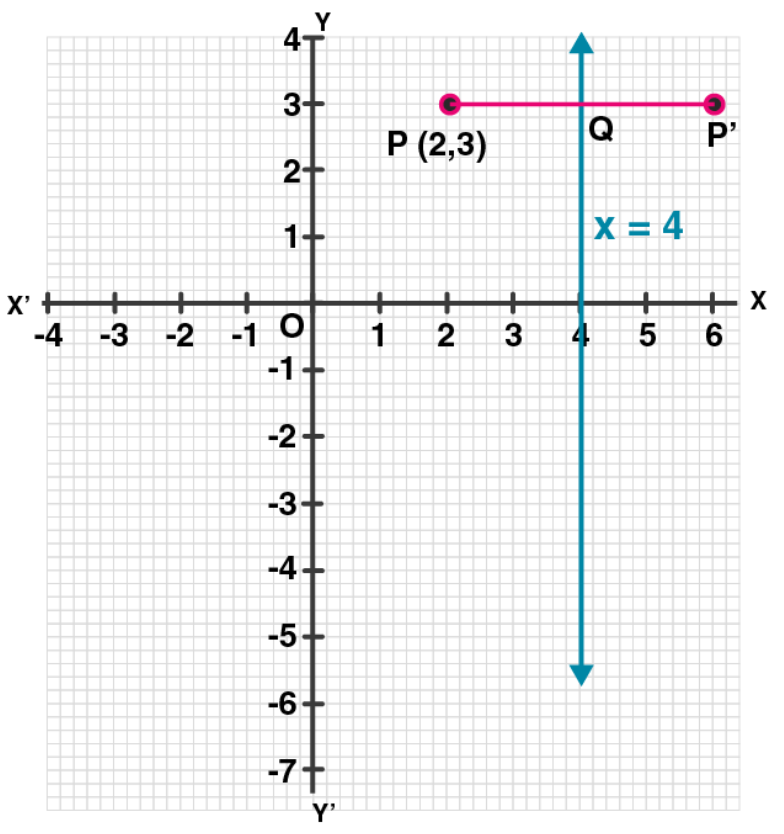(ii) The steps are:

(a) Draw axis XOX’ and YOY’ and take 1 cm = 1 unit

(b) Plot point P (2, 3) on it.

(c) Draw a line x = 4 which is parallel to y-axis.

(d) From P, draw a perpendicular on x = 4, which intersects x = 4 at Q.

(e) Produce PQ to P’, such that QP’ = QP.

Thus, P’ is the reflection of P in the line x = 4

Hence, the co-ordinates of P’ are (6, 3)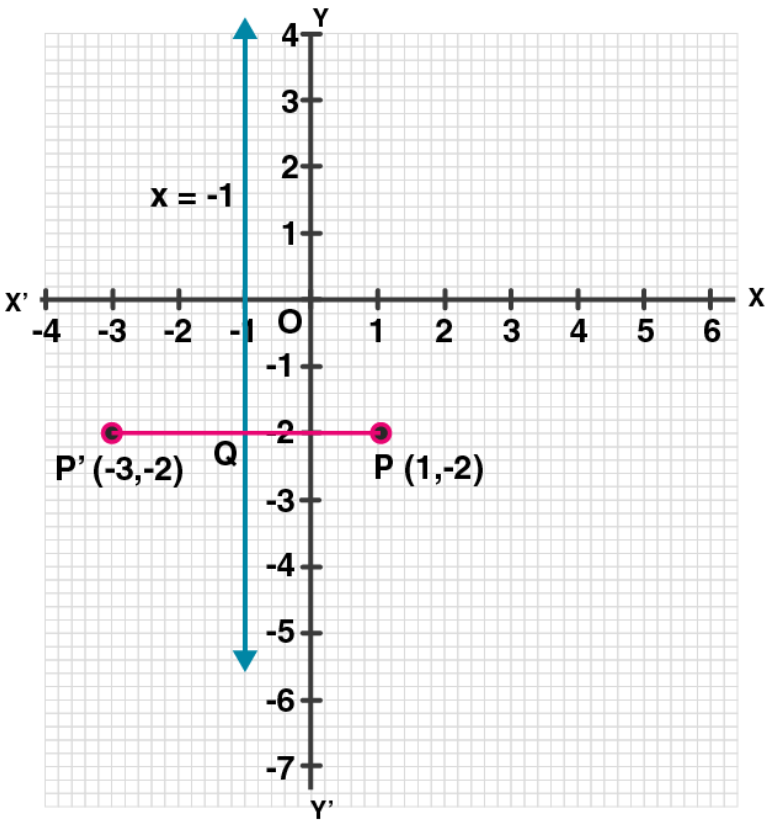8. (i) The point P (2, 4) on reflection in the line y = 1 is mapped onto P’ Find the co-ordinates of P’.
(ii) Find the image of the point P ( -3, -5) in the line y = -2.
Solution:

(i) The steps are:

(a) Draw axis XOX’ and YOY’ and take 1 cm = 1 unit.

(b) Plot point P (2, 4) on it.

(c) Draw a line y = 1, which is parallel to x-axis.

(d) From P, draw a perpendicular on y = 1 meeting it at Q.

(e) Produce PQ to P’ such that QP’ = PQ.

Therefore, P’ is the reflection of P whose co-ordinates are (2, -2).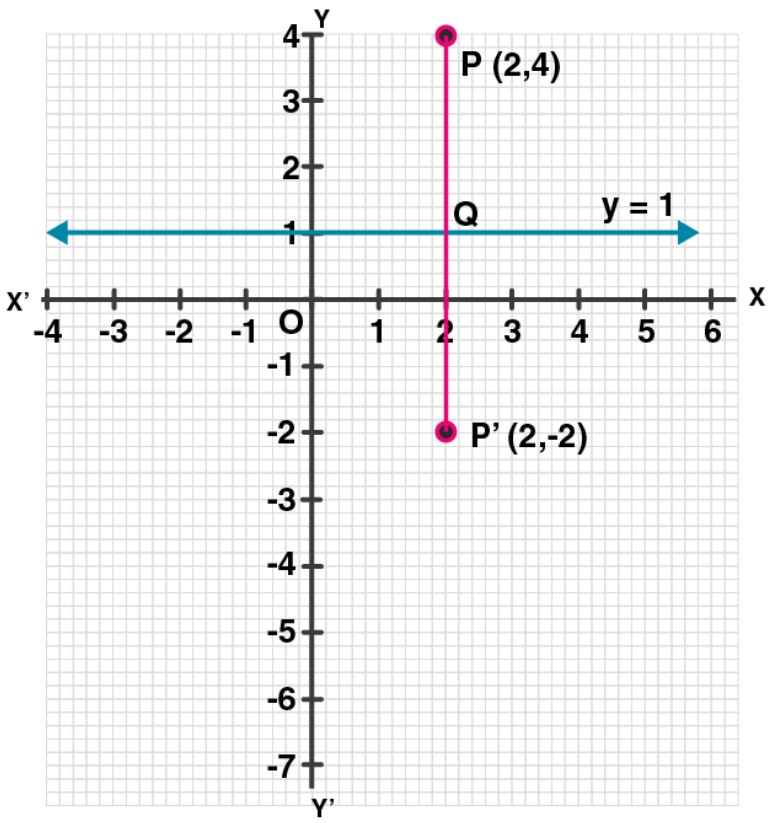(ii) The steps are:

(a) Draw axis XOX’ and YOY’ and take 1 cm = 1 unit.

(b) Plot point P (-3, -5) on it.

(c) Draw a line y = -2 which is parallel to the x-axis.

(d) From P, draw a perpendicular on y = -2 which meets it at Q.

(e) Produce PQ to P’ such that QP’ = PQ.

Therefore, P’ is the image of P, whose co-ordinates are (-3, 1).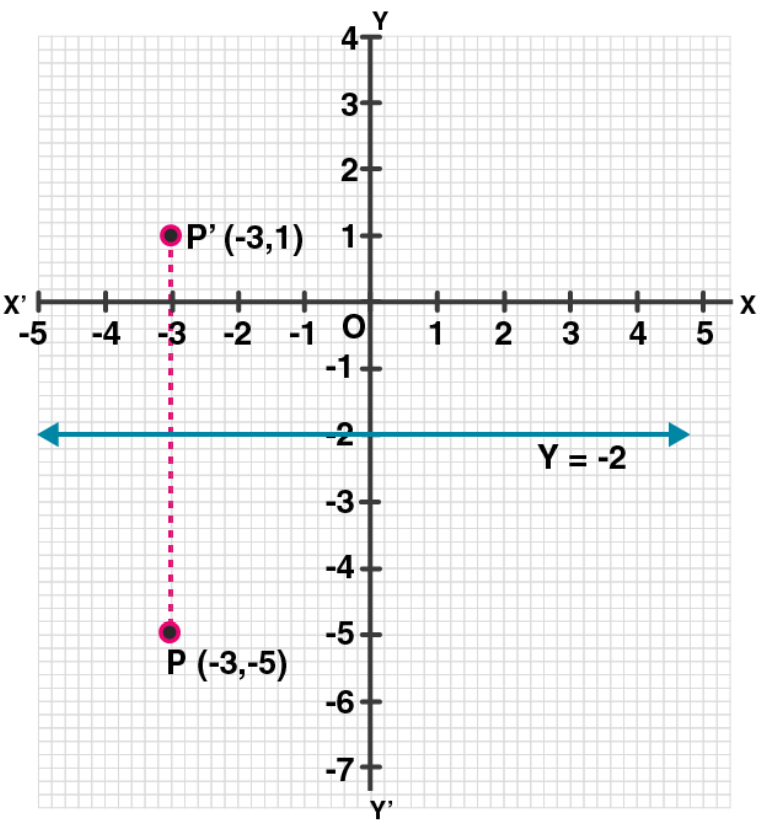9. The point P ( -4, -5) on reflection in y-axis is mapped on P’. The point P’ on reflection in the origin is mapped on P”. Find the co-ordinates of P’ and P”. Write down a single transformation that maps P onto P”.

Solution:

Given, point P (-4, -5)

And, P’ is the image of point P in y-axis

Thus, the co-ordinates of P’ will be (4, -5)

Again,

P” is the image of P’ under reflection in origin.

Thus, the co-ordinates of P’’ will be (-4, 5).

The single transformation that maps P onto P” is the x-axis.

10. Write down the co-ordinates of the image of the point (3, -2) when:
(i) reflected in the x-axis
(ii) reflected in the y-axis
(iii) reflected in the x-axis followed by a reflection in the y-axis
(iv) reflected in the origin.
Solution:

The co-ordinates of the given point are (3, -2).

Now,

(i) Co-ordinates of the image reflected in x- axis will be (3, 2)

(ii) Co-ordinates of the image reflected in y- axis will be (-3, -2)

(iii) Co-ordinates of the point reflected in x- axis followed by reflection in the y-axis will be (-3, 2)

(iv) Co-ordinates of the point reflected in the origin will be (-3, 2).

11. Find the co-ordinates of the image of (3, 1) under reflection in x-axis followed by a reflection in the line x = 1.
Solution: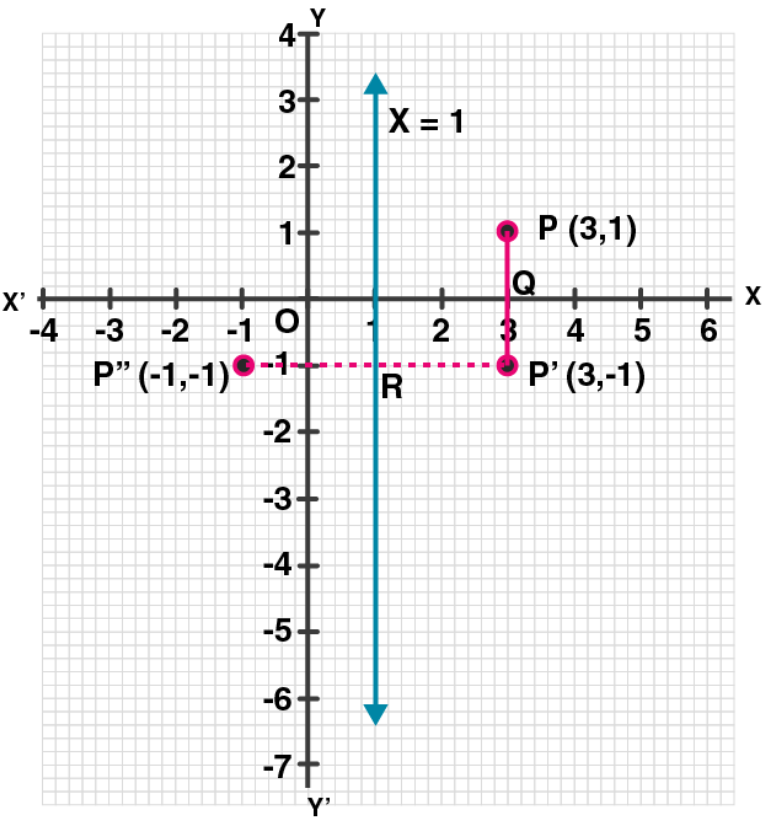The steps are:

(i) Draw axis XOX’ and YOY’ taking 1 cm = 1 unit.

(ii) Plot a point P (3, 1).

(iii) Draw a line x = 1, which is parallel to y-axis.

(iv) From P, draw a perpendicular on x-axis meeting it at Q.

(v) Produce PQ to P’ such that QP’ = PQ, then

P’ is the image of P is x-axis. Then co-ordinates of P’ will be (3, -1)

(vi) From P’, draw a perpendicular on x = 1 meeting it at R.

(vii) Produce P’R to P” such that RP” = P’R

Thus, P” is the image of P’ in the line x = 1

Hence, the co-ordinates of P” are (-1, -1)

12. If P’ (-4, -3) is the image of a point P under reflection in the origin, find
(i) the co-ordinates of P.
(ii) the co-ordinates of the image of P under reflection in the line y = -2.
Solution:

(i) Given, reflection of P is P’ (-4, -3) in the origin

Thus, the co-ordinates of P will be (4, 3)

Now,

Draw a line y = -2, which is parallel to x-axis

(ii) From P, draw a perpendicular on y = -2 meetings it at Q

Produce PQ to P” such that QP” = PQ

Thus, P” will the image of P in the line y = -2

Hence, the co-ordinates of P” will be (4, -7).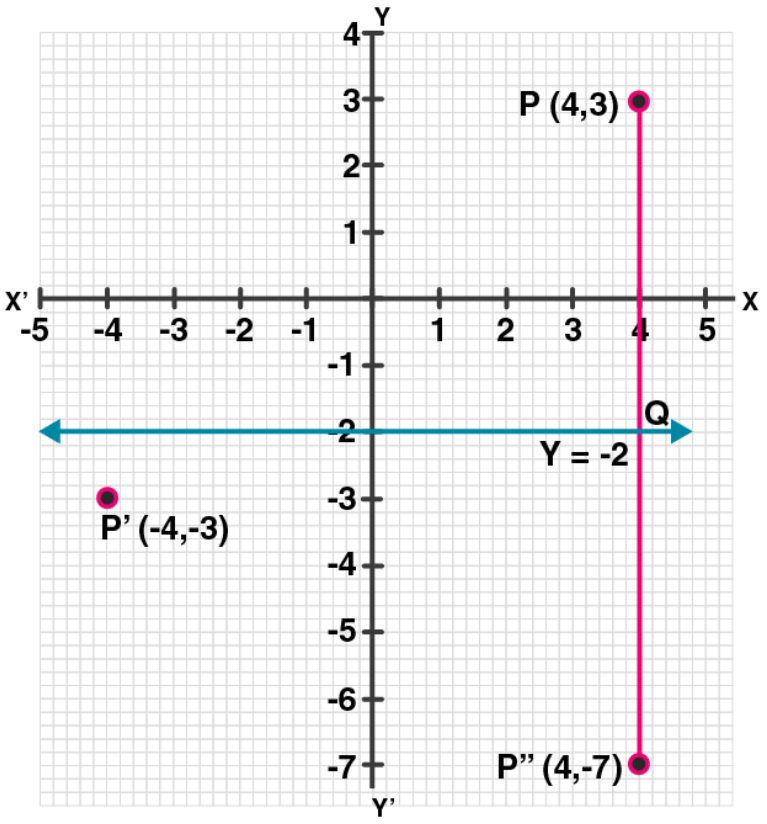13. A point P (a, b) is reflected in the x-axis to P’ (2, -3), write down the values of a and b. P” is the image of P, when reflected in the y-axis. Write down the co-ordinates of P”. Find the co-ordinates of P”, when P is reflected in the line parallel to y-axis such that x = 4.
Solution:

Given,

P’ (2, -3) is the reflection of P (a, b) in the x-axis

Hence, the co-ordinates of P’ will be (a, – b) but P’ is (2, -3)

On comparing, we get a = 2, b = 3

Thus, the co-ordinates of P will be (2, 3)

And,

P” is the image of P when reflected in y-axis

Hence, the co-ordinate of P” will be ( – 2, 3)

Now, draw a line x = 4, which is parallel to y-axis

As P’” is the image of P when it is reflected in the line x = 4,

So, P’” is its reflection.

Thus, the co-ordinates of P”’ will be (6, 3).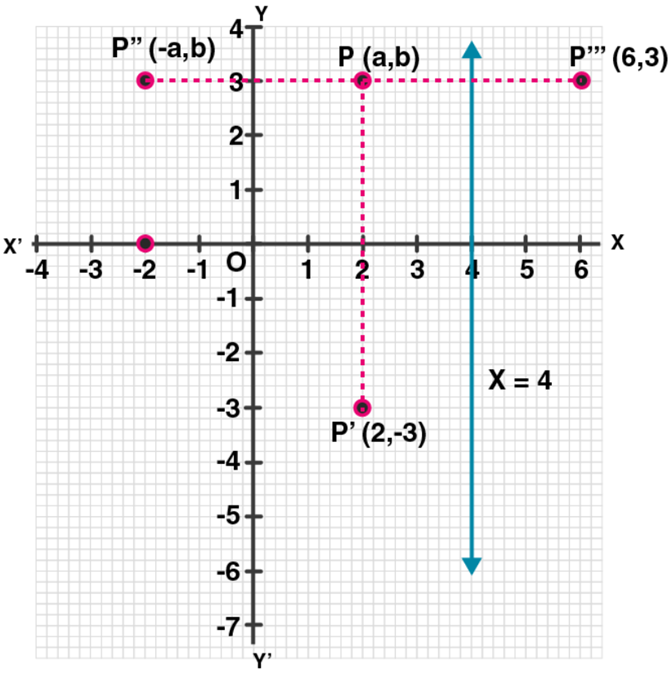14. (i) Point P (a, b) is reflected in the x-axis to P’ (5, -2). Write down the values of a and b.
(ii) P” is the image of P when reflected in the y-axis. Write down the co-ordinates of P”.
(iii) Name a single transformation that maps P’ to P”.
Solution:

(i) Image of P (a, b) reflected in the x-axis to P’ (5, -2)

So, the co-ordinates of P will be (5, 2)

Hence, a = 5 and b = 2

(ii) P” is the image of P when reflected in the y-axis

Thus, its co-ordinates will be (-5, -2).

(iii) The single transformation that maps P’ to P” is the origin.

15. Points A and B have co-ordinates (2, 5) and (0, 3). Find
(i) the image A’ of A under reflection in the x-axis.
(ii) the image B’ of B under reflection in the line AA’.
Solution:

Given, co-ordinates of A are (2, 5) and of B are (0, 3)

(i) Co-ordinates of A’, the image of A reflected in the x-axis will be (2, -5)

(ii) Co-ordinates of B’, the image of B under reflection in the line AA’ will be (4, 3).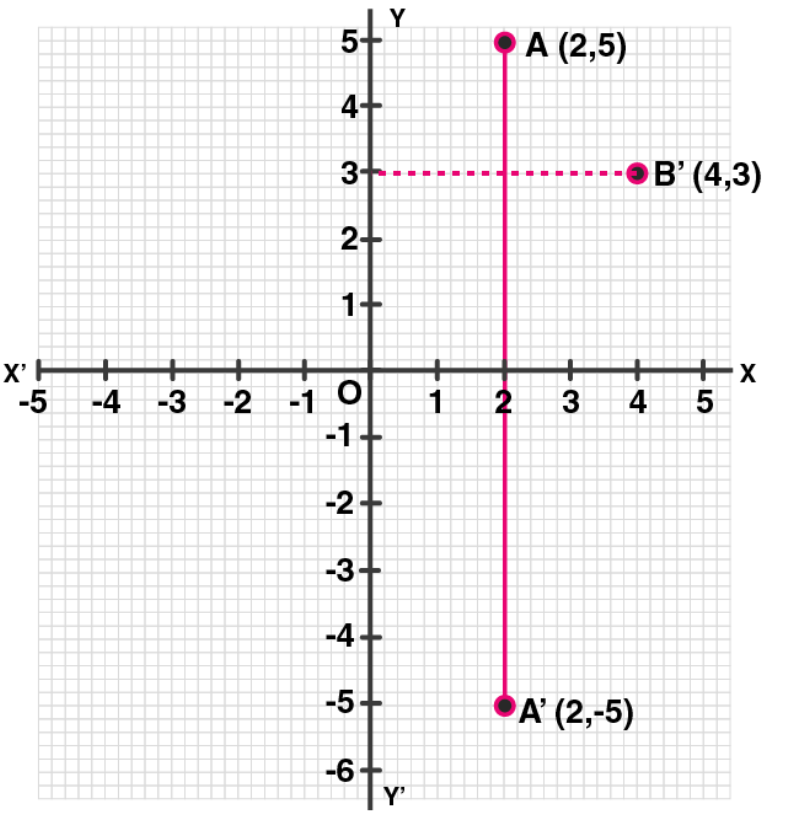16. Plot the points A (2, -3), B (-1, 2) and C (0, -2) on the graph paper. Draw the triangle formed by reflecting these points in the x-axis. Are the two triangles congruent?

Solution:

The points A (2, -3), B (-1, 2) and C(0, -2) has been plotted on the graph paper as shown and are joined to form a triangle ABC.

Hence, the co-ordinates of the images of A, B and C reflected in x-axis will be A’ (2, 3), B’ (-1, -2), C’ (0, 2) respectively.

And, these are joined to from another ∆ A’B’C’

Yes, these two triangles are congruent.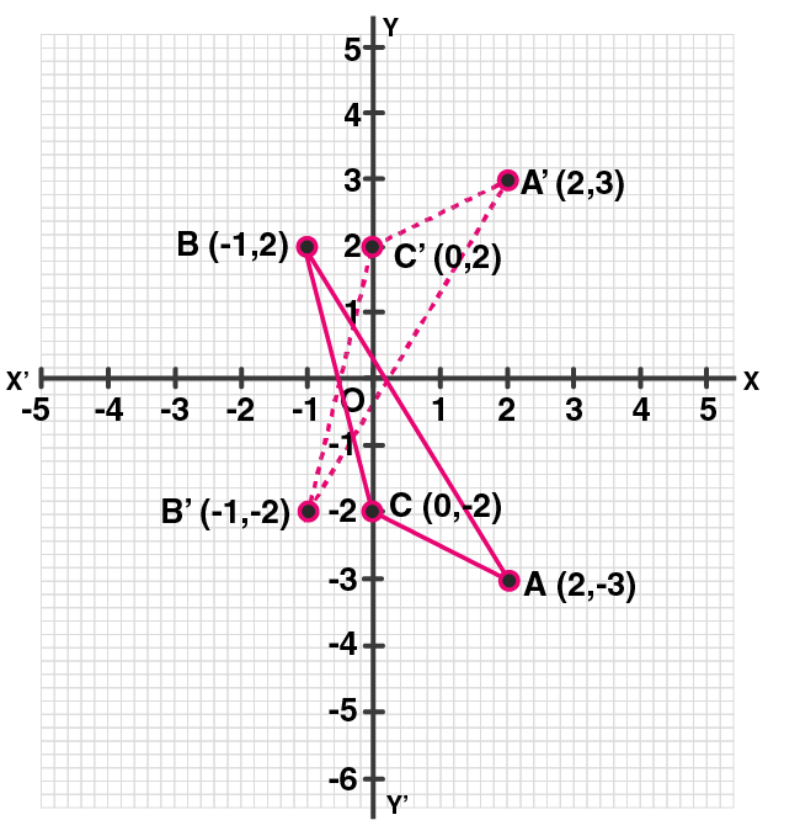17. The points (6, 2), (3, -1) and (-2, 4) are the vertices of a right-angled triangle. Check whether it remains a right-angled triangle after reflection in the y-axis.
Solution:

Let A (6, 2), B (3, -1) and C (-2, 4) be the points of a right-angled triangle

Then,

The co-ordinates of the images of A, B, C reflected in y-axis will be:

A’ (-6, 2), B’ (-3, -1) and C’ (2, 4).

Hence, by joining these points

We see that ∆A’B’C’ is also a right-angled triangle.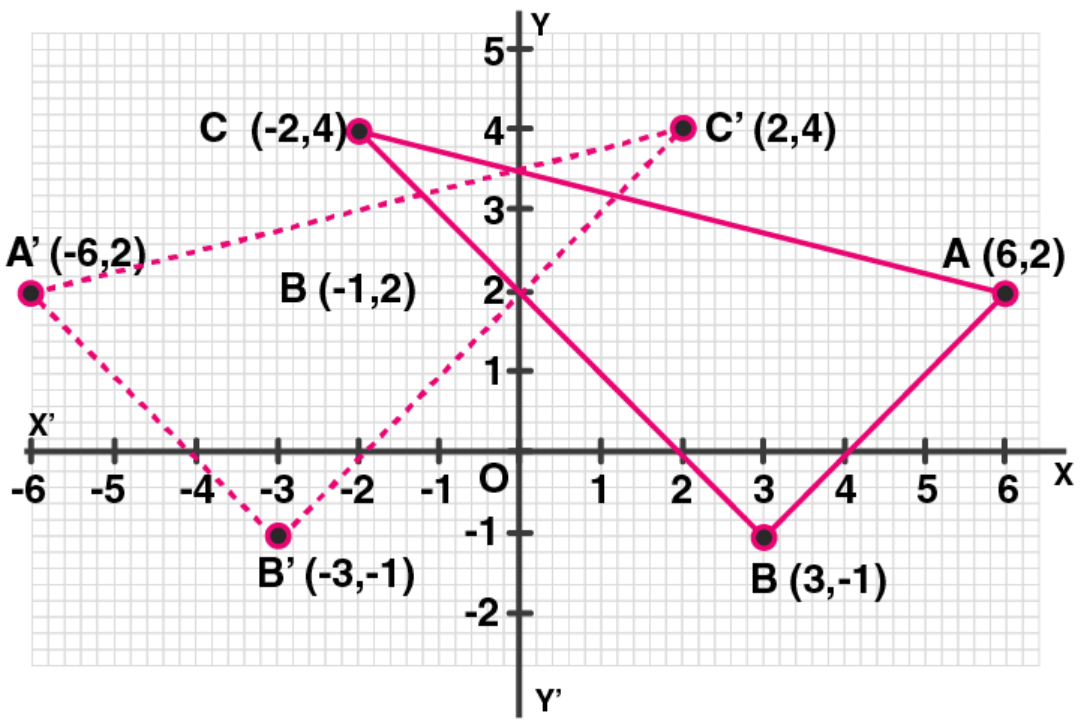18. The triangle ABC where A (1, 2), B (4, 8), C (6, 8) is reflected in the x-axis to triangle A’ B’ C’. The triangle A’ B’ C’ is then reflected in.the origin to triangle A”B”C” Write down the co-ordinates of A”, B”, C”. Write down a single transformation that maps ABC onto A” B” C”.
Solution:

Given,

The co-ordinates of ∆ ABC are A (1, 2) B (4, 8), C (6, 8)

These vertices are reflected in x- axis as A’, B’ and C’.

Hence, their co-ordinates are A’ (1, -2), B’ (4, -8) and C’ (6, -8).

Now,

A’, B’ and C’ are again reflected in origins to form an ∆A”B”C”.

Hence, the co-ordinates will be A” (-1, 2), B” (-4, 8) and C” (-6, 8)

The single transformation that maps ABC onto A” B” C” is y-axis.

19. The image of a point P on reflection in a line l is point P’. Describe the location of the line l.
Solution:

The line will be the right bisector of the line segment joining P and P’.

20. Given two points P and Q, and that (1) the image of P on reflection in the y-axis is the point Q and (2) the midpoint of PQ is invariant on reflection in x-axis. Locate: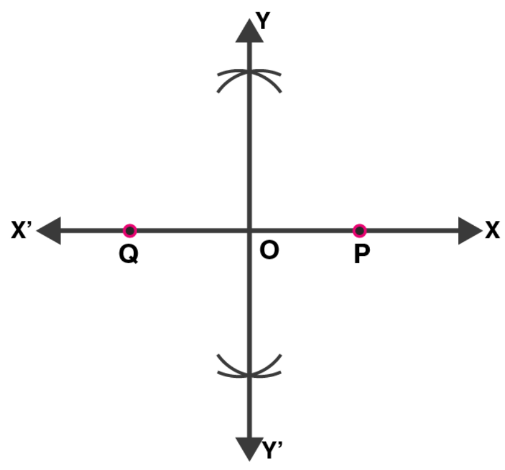(i) the x-axis
(ii) the y-axis and
(iii) the origin.
Solution:

Given, Q is the image of P on reflection in y-axis and mid-point of PQ is invariant on reflection in x-axis

(i) x-axis will be the line joining the points P and Q.

(ii) The line perpendicular bisector of line segment PQ is the y-axis.

(iii) The origin will be the mid-point of line segment PQ.

21. The point (-3, 0) on reflection in a line is mapped as (3, 0) and the point (2, -3) on reflection in the same line is mapped as (-2, -3).
(i) Name the mirror line.
(ii) Write the co-ordinates of the image of (-3, -4) in the mirror line.
Solution:

Given,

The point (-3,0) is the image of point (3, 0) and point (2, -3) is image of point (-2, -3) reflected on the same line.

(i) Clearly, it’s seen that the mirror line will be y-axis.

(ii) The co-ordinates of the image of the point (-3, -4) reflected in the same line i.e. y-axis will be (3, -4).

22. Use graph paper for this (take 2 cm = 1 unit along both x and y-axis). ABCD is a quadrilateral whose vertices are A (2, 2), B (2, -2), C (0, -1) and D (0, 1).

(i) Reflect quadrilateral ABCD on the y-axis and name it as A’B’CD.

(ii) Write down the coordinates of A’ and B’.

(iii) Name two points which are invariant under the above reflection.

(iv) Name the polygon A’B’CD.

Solution: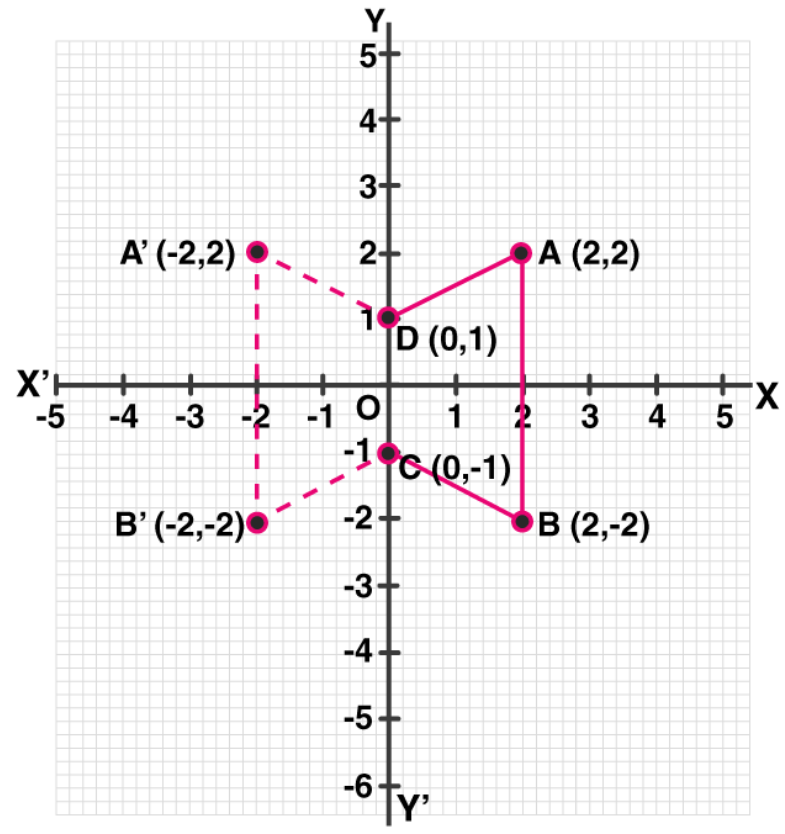(i) Quadrilateral ABCD is reflected on the y-axis and named as A’B’CD.

(ii) As A’ is the reflection of A (2, 2) about the line x = 0 (y-axis)

Thus, the co-ordinates of A’ are (– 2, 2).

And, as B’ is the reflection of B (2, -2) about the line x = 0 (y-axis)

Thus, the coordinates of B’ are (-2, -2).

(iii) Points C (0, -1) and D (0, 1) are invariant under the above reflection.

(iv) The polygon A’B’CD is a trapezium since A’B’ || CD.

23. Use a graph sheet for this question. Take 1 cm = 1 unit along both x and y-axis.

(i) Plot the point: A (0, 5), B (3, 0), C (1, 0) and D (1, -5).

(ii) Reflect the points B, C and D on the y-axis and name them as B’, C’ and D’ respectively.

(iii) Write down the coordinates of B’, C’ and D’.

(iv) Join the points A, B, C, D, D’, C’, B’, A in order and give a name to the closed figure ABCDD’C’B’.

Solution: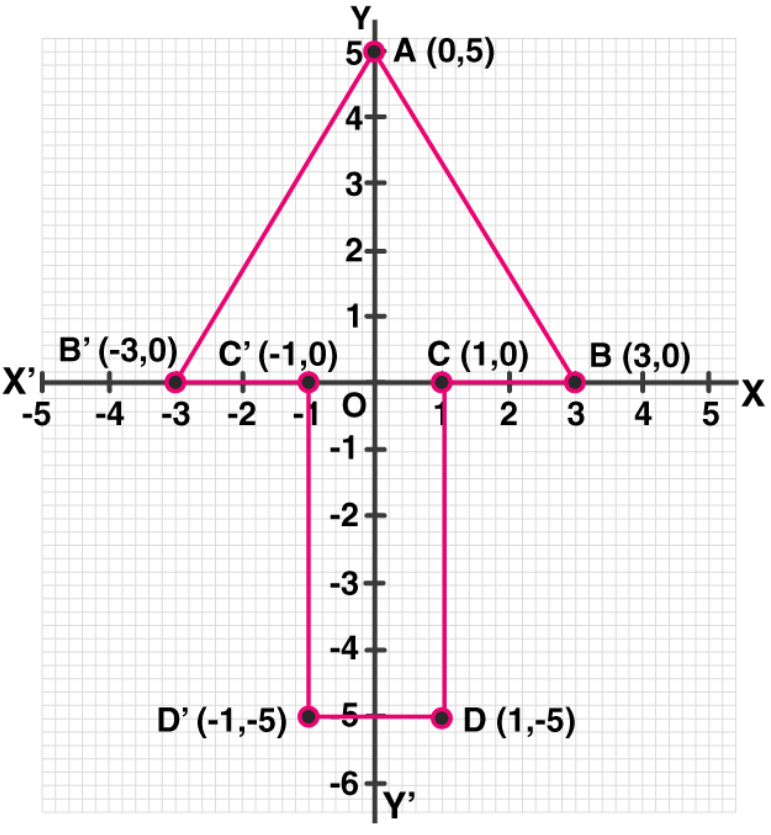(i) Points A (0, 5), B (3, 0), C (1, 0) and D (1, -5) are plotted on the graph sheet.

(ii) Points B, C and D are reflected on the y-axis and named as B’, C’ and D’ respectively.

(iii) The coordinates of B’ are (-3, 0), C’ (-1, 0) and D’ (-1, -5).

(iv) Points A, B, C, D, D’, C’, B’, A are joined in order and the closed figure comes out to be an arrow mark (Or a heptagon).

24. Use graph paper for this question.
(i) The point P (2, -4) is reflected about the line x = 0 to get the image Q. Find the co-ordinates of Q.
(ii) Point Q is reflected about the line y = 0 to get the image R. Find the co-ordinates of R.
(iii) Name the figure PQR.
(iv) Find the area of figure PQR.
Solution:

(i) As the point Q is the reflection of the point P (2, -4) in the line x = 0,

Thus, the co-ordinates of Q are (2, 4).

(ii) As R is the reflection of Q (2, 4) about the line y = 0,

Thus, the co-ordinates of R are (– 2, 4).

(iii) Figure PQR is the right-angled triangle PQR.

(iv) Area of ∆ PQR = ½ x QR x PQ

= ½ x 4 x 8

= 16 sq. units.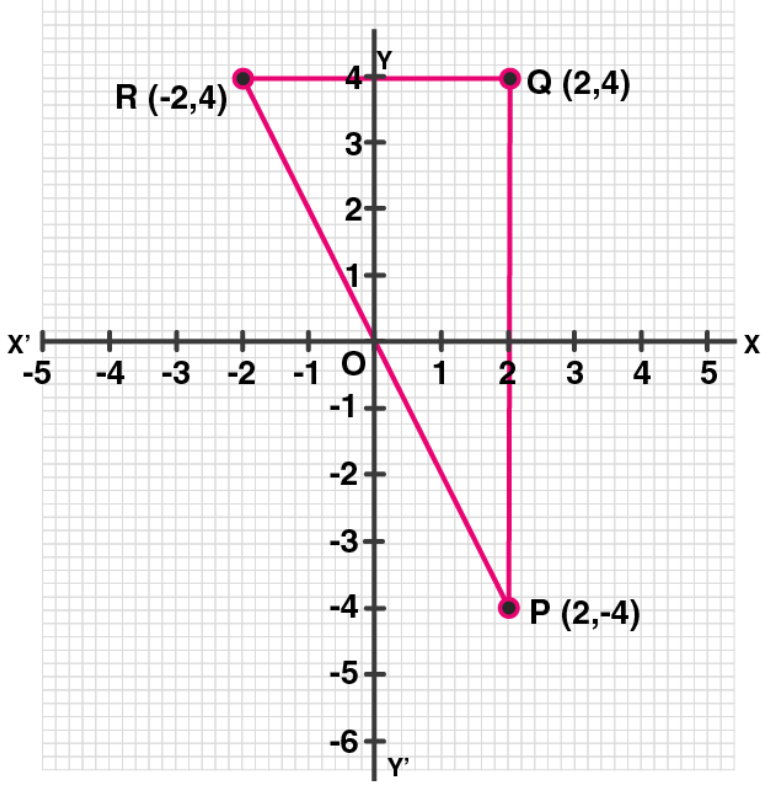25. Using a graph paper, plot the points A (6, 4) and B (0, 4).
(i) Reflect A and B in the origin to get the images A’ and B’.
(ii) Write the co-ordinates of A’ and B’.
(iii) State the geometrical name for the figure ABA’B’.
(iv) Find its perimeter.
Solution:

Points A (6, 4) and B (0, 4) are plotted on a graph paper.

(i) A and B are reflected in the origin to get images A’ and B’

(ii) Hence,

The co-ordinates of A’ are (-6, -4)

The co-ordinates of B’ are (0, -4)

(iii) The geometrical name for ABA’B’ is parallelogram

(iv) From the figure in graph paper, we see that

Length of AB = A’B’ = 6 units

And, BB’ = 8 units

In ∆ ABB’, by Pythagoras theorem

(AB’)2 = AB2 + (BB’)2

= 62 + 82

= 36 + 64 = 100

AB’ = √100 = 10 units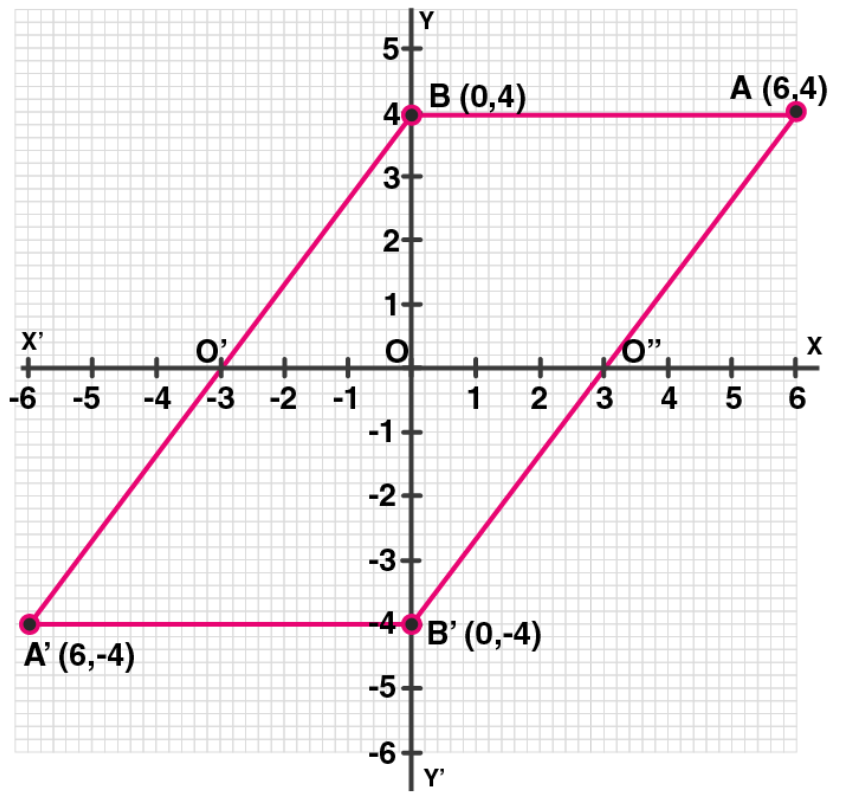Hence, the perimeter of ABA’B’ = (6 + 10 + 6 + 10) = 32 units

26. Use graph paper to answer this question
(i) Plot the points A (4, 6) and B (1, 2).
(ii) If A’ is the image of A when reflected in x-axis, write the co-ordinates of A’.
(iii) If B’ is the image of B when B is reflected in the line AA’, write the co-ordinates of B’.
(iv) Give the geometrical name for the figure ABA’B’.
Solution:

(i) Plotting the points A (4, 6) and B (1, 2) on the given graph.

(ii) The co-ordinates of the image of A when reflected in axis are A’(4, -6)

(iii) The co-ordinates of the image of B when reflected in the line AA’ are B’ = (7, 2)

(iv) It’s seen that in the quadrilateral ABA’B’, we have

AB = AB’ and A’B = A’B’

Thus, ABA’B’ is a kite.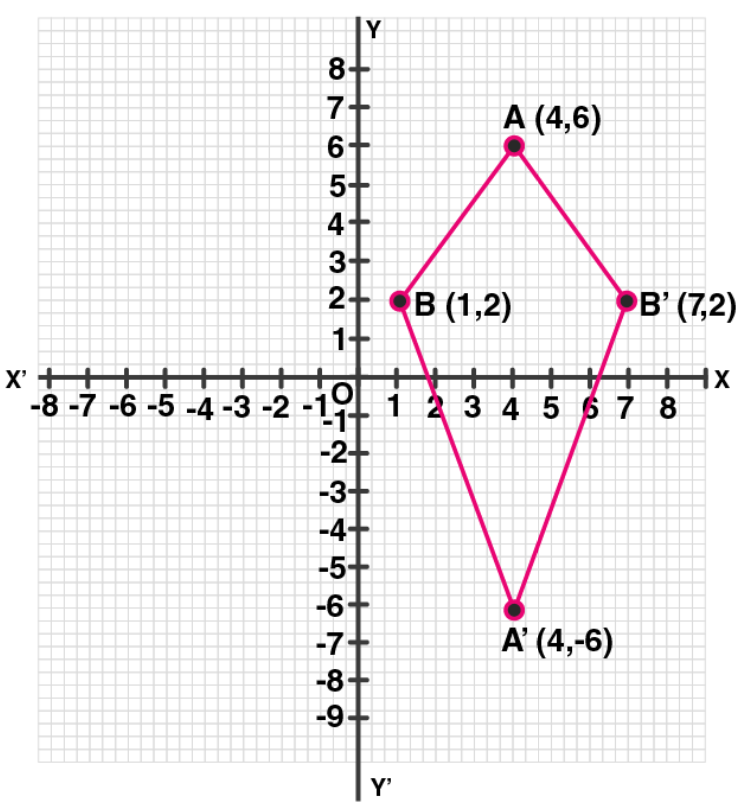27. The points A (2, 3), B (4, 5) and C (7, 2) are the vertices of ∆ABC.
(i) Write down the co-ordinates of A1, B1, C1 if ∆ A1B1C1 is the image of ∆ ABC when reflected in the origin.
(ii) Write down the co-ordinates of A2, B2, C2 if ∆ A2B2C2 is the image of ∆ ABC when reflected in the x-axis.
(iii) Assign the special name to the quadrilateral BCC2B2 and find its area.

Solution:

Given, points A (2, 3), B (4, 5) and C (7, 2) are the vertices of ∆ ABC.

And A1, B1 and C1 are the images of A, B and C reflected in the origin.

(i) Hence,

Co-ordinates of A1 = (-2, -3)

Co-ordinates of B1 (-4, -5) and

Co-ordinates of C1 (-7, -2).

(ii) Now,

Co-ordinates of A2, B2 and C2 the images of A, B and C when reflected in x-axis are:

A2 (2, – 3), B2 (4, – 5), C2 (7, – 2)

(iii) The quadrilateral formed by joining the points, BCC2B2 is an isosceles trapezium and its area is

= ½ (BB2 + CC2) x 3

= ½ (10 + 4) x 3

= ½ x 14 x 3

= 21 sq. units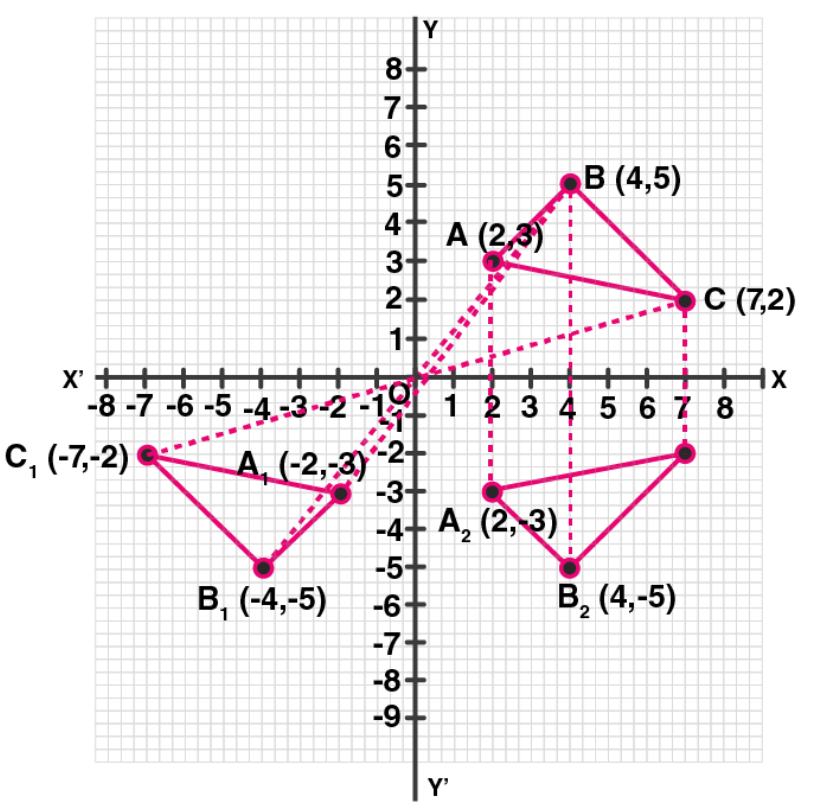28. The point P (3, 4) is reflected to P’ in the x-axis and O’ is the image of O (origin) in the line PP’. Find:
(i) the co-ordinates of P’ and O’,
(ii) the length of segments PP’ and OO’.
(iii) the perimeter of the quadrilateral POP’O’.
Solution:

Given,

P’ is the image of P (3, 4) reflected in x- axis and O’ is the image of O the origin in the line P’P.

(i) Hence, co-ordinates of P’ are (3, -4) and co-ordinates of O’ reflected in PP’ are (6, 0)

(ii) Length of PP’ = 8 units and OO’ = 6 units

(iii) Perimeter of POP’O’ is (4 x OP) units.

Let Q be the point of intersection of diagonals OO’ and PP’.

So, OQ = 3 units and OP = 4 units

Hence,

OP = √[(OQ)2 + (PQ)2] = √ (32 + 42) = √(9 + 16) = √25 = 5 units

Thus, the perimeter of POP’O’ = 4 x 5 = 20 units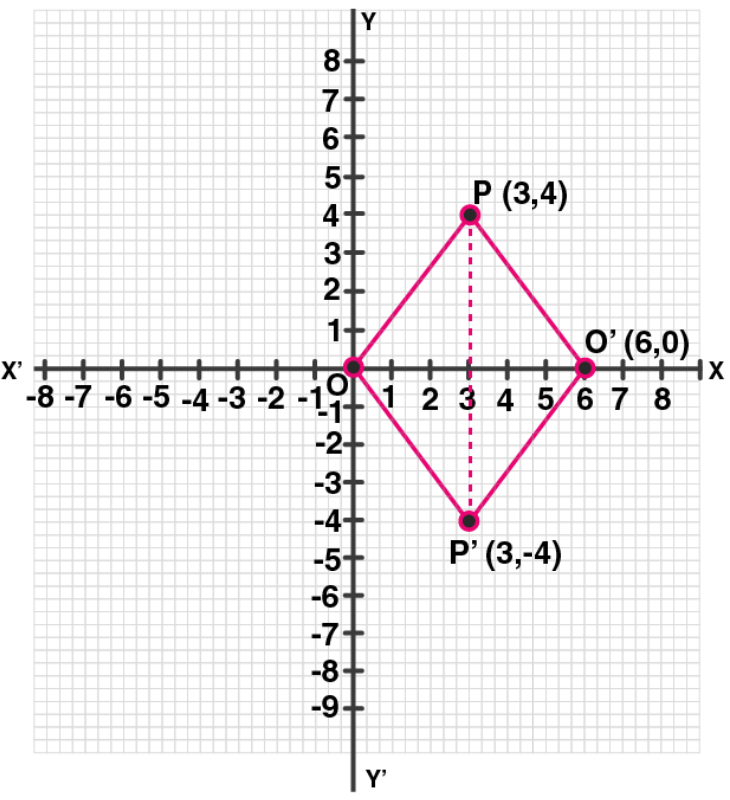29. Use a graph paper for this question. (Take 10 small divisions = 1 unit on both axes). P and Q have co-ordinates (0, 5) and (-2, 4).
(i) P is invariant when reflected in an axis. Name the axis.
(ii) Find the image of Q on reflection in the axis found in (i).
(iii) (0, k) on reflection in the origin is invariant. Write the value of k.
(iv) Write the co-ordinates of the image of Q, obtained by reflecting it in the origin followed by a reflection in x-axis.
Solution:

Given, two points P (0, 5) and Q (-2, 4)

(i) As the abscissa of P is 0. It is invariant when is reflected in y-axis.

(ii) Let Q’ be the image of Q on reflection in y-axis.

Thus, the co-ordinate of Q’ will be (2, 4)

(iii) (0, k) on reflection in the origin is invariant.

So, the co-ordinates of image will be (0, 0)

Hence, k = 0

(iv) The reflection of Q in the origin is the point Q” and its co-ordinates will be (2, – 4) and reflection of Q” (2, – 4) in x-axis is (2, 4) which is the point Q’.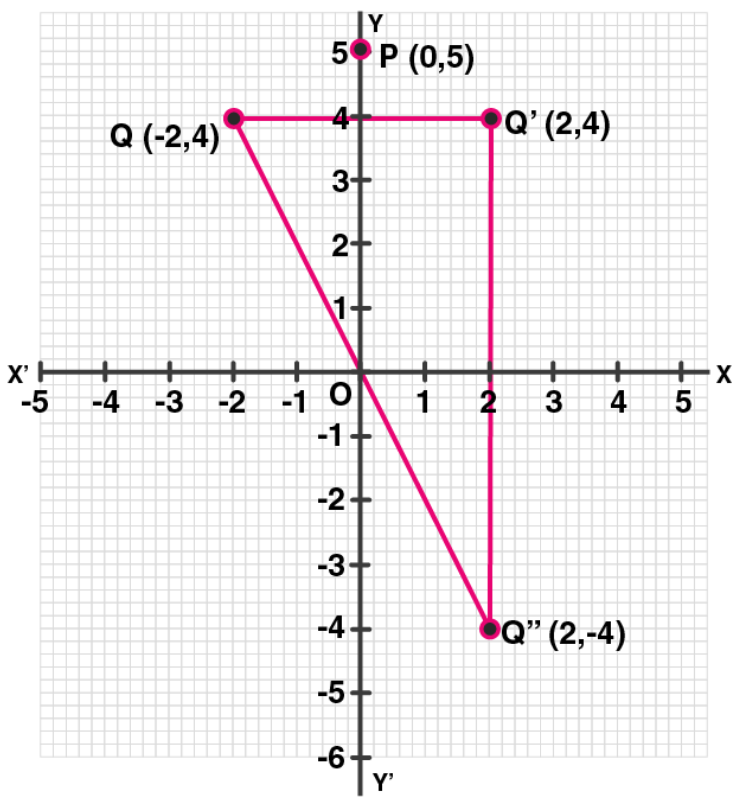Chapter Test

1. The point P (4, – 7) on reflection in x-axis is mapped onto P’. Then P’ on reflection in the y-axis is mapped onto P”. Find the co-ordinates of P’ and P”. Write down a single transformation that maps P onto P”.

Solution:

Given,

P’ is the image of P (4, -7) reflected in x-axis

Thus, the co-ordinates of P’ are (4, 7)

Again P” is the image of P’ reflected in y-axis

Hence, the co-ordinates of P” are (-4, 7)

Therefore, single transformation that maps P and P” is in the origin.

2. The point P (a, b) is first reflected in the origin and then reflected in the y-axis to P’. If P’ has co-ordinates (3, – 4), evaluate a, b

Solution:

The co-ordinates of image of P (a, b) reflected in origin are (-a, -b).

Again, the co-ordinates of P’ which is image of the above point (-a, -b) reflected in the y-axis are (a, -b).

But the co-ordinates of P’ are (3, -4)

Thus, a = 3 and -b = -4 ⇒ b = 4

3. A point P (a, b) becomes (– 2, c) after reflection in the x-axis, and P becomes (d, 5) after reflection in the origin. Find the values of a, b, c and d.

Solution:

Given, point P (a, b) and the image of P (a, b) after reflected in the x-axis be (a, -b)

But it is given as (-2, c)

Thus, a = -2, c = -b

Next,

If P is reflected in the origin, then its co-ordinates will be (-a, -b)

But it is given as (d, 5)

Thus,

-b = 5 ⇒ b = -5,

d = -a = -(-2) = 2,

c = -b = -(-5) = 5

Thus,

a = -2, b = -5, c = 5 and d = 2

4. A (4, – 1), B (0, 7) and C (– 2, 5) are the vertices of a triangle. ∆ ABC is reflected in the y-axis and then reflected in the origin. Find the co-ordinates of the final images of the vertices.

Solution:

Given, A (4, -1), B (0, 7) and C (-2, 5) are the vertices of ∆ABC.

∆ABC after reflecting in y-axis, the co-ordinates of points will be A’ (-4, -1), B’ (0, 7), C’ (2, 5).

Again, when ∆A’B’C’ reflecting in origin:

The co-ordinates of the images of the vertices will be A” (4, 1), B” (0, -7), C” (-2, -5).

5. The points A (4, – 11), B (5, 3), C (2, 15), and D (1, 1) are the vertices of a parallelogram. If the parallelogram is reflected in the y-axis and then in the origin, find the co-ordinates of the final images. Check whether it remains a parallelogram. Write down a single transformation that brings the above change.

Solution:

Given, points A (4, -11), B (5, 3), C (2, 15) and D (1, 1) are the vertices of a parallelogram.

After reflecting in y-axis, the images of these points will be

A’ ( -4, 11), B’ (-5, 3), C (-2, 15) and D’ (-1, 1).

Again, reflecting these points in origin, the image of these points will be A” (4, -11), B” (5, -3), C” (2, -15) and D” (0, -1).

Yes, the reflection of a single transformation is in the x-axis.

6. Use a graph paper for this question (take 2 cm = 1 unit on both x and y axes).

(i) Plot the following points: A (0, 4), B (2, 3), C (1, 1) and D (2, 0).

(ii) Reflect points B, C, D on y-axis and write down their coordinates. Name the images as B’, C’, D’ respectively.

(iii) Join points A, B, C, D, D’, C’, B’ and A in order, so as to form a closed figure. Write down the equation of line of symmetry of the figure formed.

Solution:

(i) On graph: A (0, 4), B (2, 3), C (1, 1) and D (2, 0)

(ii) Point after reflection on y-axis are B’ = (-2, 3), C’ = (-1, 1) and D’ = (-2, 0)

(iii) The points A, B, C, D, D’, C’, B’ and A in order to form a closed figure.

Hence, the equation of the line of symmetry is x = 0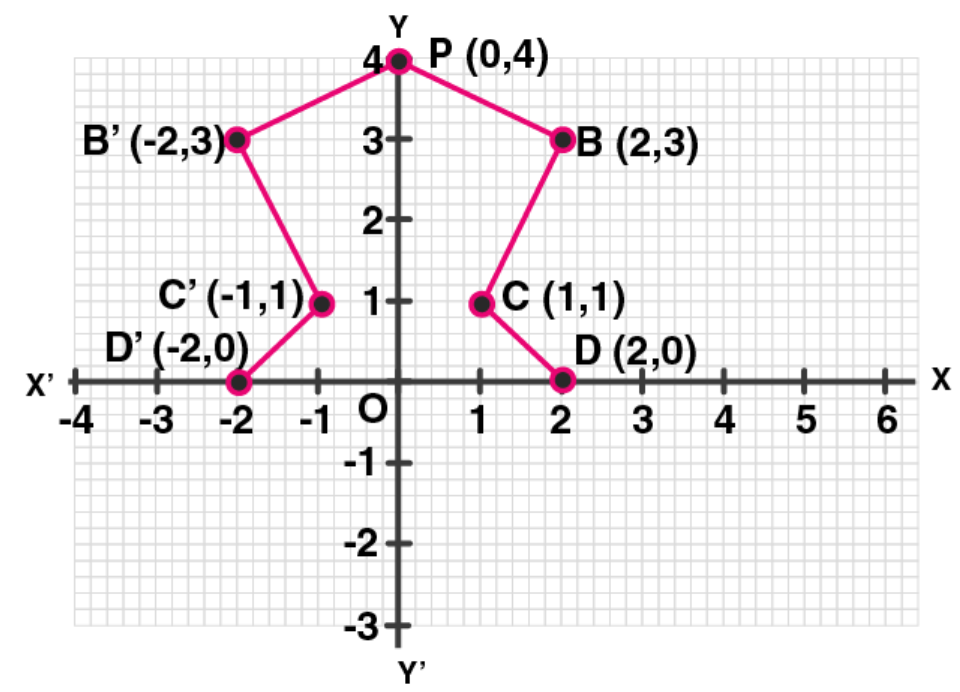7. The triangle OAB is reflected in the origin O to triangle OA’B’. A’ and B’ have coordinates ( – 3, – 4) and (0, – 5) respectively.

(i) Find the co-ordinates of A and B.

(ii) Draw a diagram to represent the given information.

(iii) What kind of figure is the quadrilateral ABA’B’?

(iv) Find the coordinates of A”, the reflection of A in the origin followed by reflection in the y-axis.

(v) Find the co-ordinates of B”, the reflection of B in the x-axis followed by reflection in the origin.

Solution:

Given,

∆ OAB is reflected in the origin O to ∆ OA’B’,

And the co-ordinates of A’ = (-3, -4) and B’ = (0, -5).

(i) Hence, the co-ordinates of A will be (3, 4) and of B will be (0, 5).

(ii) The diagram representing the given information has been drawn here.

(iii) The figure in the diagram is a rectangle.

(iv) The co-ordinates of B’, the reflection of B is the x-axis is (0, -5) and co-ordinates of B”, the reflection in origin of the point (0, -5) will be (0, 5).

(v) The co-ordinates of the points, the reflection of A in the origin are (-3, -4) and coordinates of A”, the reflected in y-axis of the point (-3, – 4) are (3, -4).